8.3

### 32D Renderers

 (require plot) package: plot-gui-lib

#### 3.12D Renderer Function Arguments

Functions that return 2D renderers always have these kinds of arguments:
• Required (and possibly optional) arguments representing the graph to plot.

• Optional keyword arguments for overriding calculated bounds, with the default value #f.

• Optional keyword arguments that determine the appearance of the plot.

• The optional keyword argument #:label, which specifies the name of the renderer in the legend.

We will take function, perhaps the most commonly used renderer-producing function, as an example.

Graph arguments. The first argument to function is the required f, the function to plot. It is followed by two optional arguments x-min and x-max, which specify the renderer’s x bounds. (If not given, the x bounds will be the plot area x bounds, as requested by another renderer or specified to plot using #:x-min and #:x-max.)

These three arguments define the graph of the function f, a possibly infinite set of pairs of points x,(f x). An infinite graph cannot be plotted directly, so the renderer must approximately plot the points in it. The renderer returned by function does this by drawing lines connected end-to-end.

Overriding bounds arguments. Next in function’s argument list are the keyword arguments #:y-min and #:y-max, which override the renderer’s calculated y bounds if given.

Appearance arguments. The next keyword argument is #:samples, which determines the quality of the renderer’s approximate plot (higher is better). Following #:samples are #:color, #:width, #:style and #:alpha, which determine the color, width, style and opacity of the lines comprising the plot.

In general, the keyword arguments that determine the appearance of plots follow consistent naming conventions. The most common keywords are #:color (for fill and line colors), #:width (for line widths), #:style (for fill and line styles) and #:alpha. When a function needs both a fill color and a line color, the fill color is given using #:color, and the line color is given using #:line-color (or some variation, such as #:line1-color). Styles follow the same rule.

Every appearance keyword argument defaults to the value of a parameter. This allows whole families of plots to be altered with little work. For example, setting (line-color 3) causes every subsequent renderer that draws connected lines to draw its lines in blue.

Label argument. Lastly, there is #:label. If given, the function renderer will generate a label entry that plot puts in the legend. The label argument can be a string or a pict. For most use cases, the string will be sufficient, especially since it allows using Unicode characters, and thus some mathematical notation. For more complex cases, a pict can be used, whic allows arbitrary text and graphics to be used as label entries.

Changed in version 7.9 of package plot-gui-lib: Added support for pictures for #:label

Not every renderer-producing function has a #:label argument; for example, error-bars.

#### 3.22D Point Renderers

procedure

 (points vs [ #:x-min x-min #:x-max x-max #:y-min y-min #:y-max y-max #:sym sym #:color color #:fill-color fill-color #:x-jitter x-jitter #:y-jitter y-jitter #:size size #:line-width line-width #:alpha alpha #:label label]) → renderer2d?
vs : (sequence/c (sequence/c #:min-count 2 real?))
x-min : (or/c rational? #f) = #f
x-max : (or/c rational? #f) = #f
y-min : (or/c rational? #f) = #f
y-max : (or/c rational? #f) = #f
sym : point-sym/c = (point-sym)
color : plot-color/c = (point-color)
fill-color : (or/c plot-color/c 'auto) = 'auto
x-jitter : (>=/c 0) = (point-x-jitter)
y-jitter : (>=/c 0) = (point-y-jitter)
size : (>=/c 0) = (point-size)
line-width : (>=/c 0) = (point-line-width)
alpha : (real-in 0 1) = (point-alpha)
label : (or/c string? pict? #f) = #f
Returns a renderer that draws points. Use it, for example, to draw 2D scatter plots.

The renderer sets its bounds to the smallest rectangle that contains the points. Still, it is often necessary to override these bounds, especially with randomized data. For example,
 > (parameterize ([plot-width    150] [plot-height   150] [plot-x-label  #f] [plot-y-label  #f]) (define xs (build-list 20 (λ _ (random)))) (define ys (build-list 20 (λ _ (random)))) (list (plot (points (map vector xs ys))) (plot (points (map vector xs ys) #:x-min 0 #:x-max 1 #:y-min 0 #:y-max 1))))

'(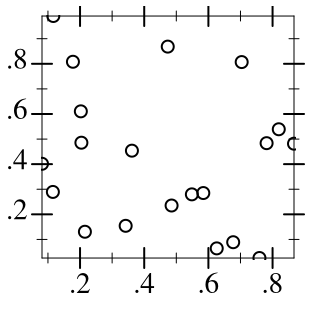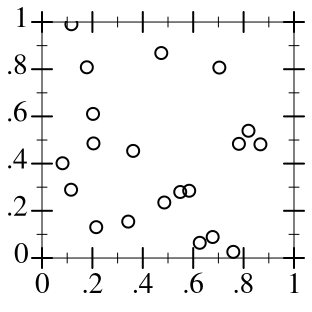)

Readers of the first plot could only guess that the random points were generated in [0,1] × [0,1].

The #:sym argument may be any integer, a Unicode character or string, or a symbol in known-point-symbols. Use an integer when you need different points but don’t care exactly what they are.

When x-jitter is non-zero, all points are translated by a random amount at most x-jitter from their original position along the x-axis. A non-zero y-jitter similarly translates points along the y-axis. Jitter is added in both directions so total spread is twice the value given. To be precise, each point p is moved to a random location inside a rectangle centered at p with width at most twice x-jitter and height at most twice y-jitter subject to the constraint that new points lie within [x-min, x-max] and [y-min, y-max] if these bounds are non-#f.

 > (plot (points (for/list ([i (in-range 1000)]) (list 0 0)) #:alpha 0.4 #:x-jitter 1 #:y-jitter 1 #:sym 'fullcircle1 #:color "blue") #:x-min -2 #:x-max 2 #:y-min -2 #:y-max 2)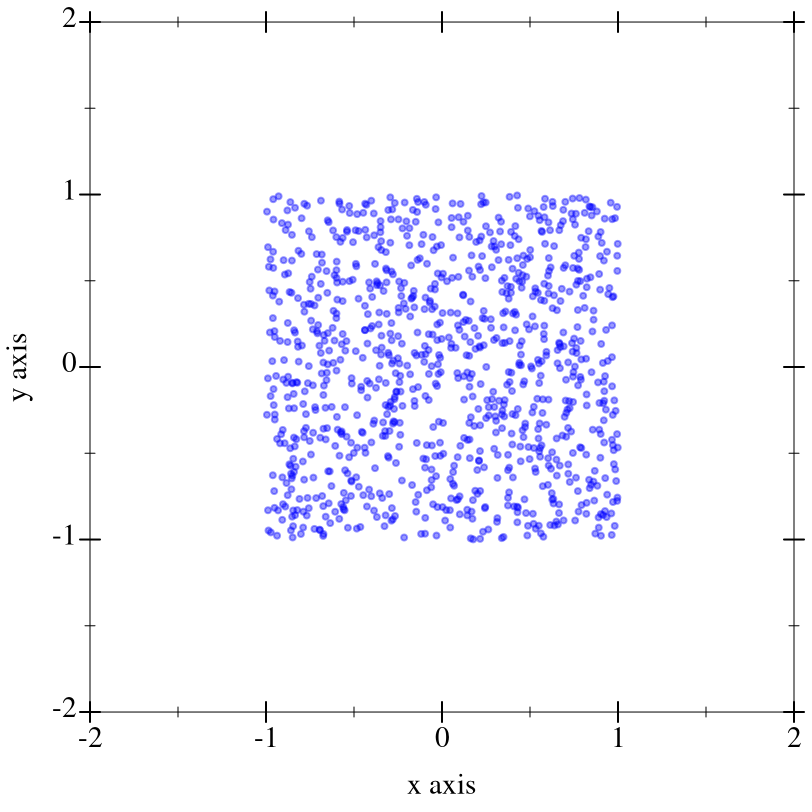Randomly moving data points is almost always a bad idea, but jittering in a controlled manner can sometimes be useful. For example:

• To highlight the size of a dense (or overplotted) sample.

• To see the distribution of 1-dimensional data; as a substitute for box or violin plots.

• To anonymize spatial data, showing i.e. an office’s neighborhood but hiding its address.

Changed in version 7.9 of package plot-gui-lib: Added support for pictures for #:label

procedure

 (vector-field f [ x-min x-max y-min y-max #:samples samples #:scale scale #:color color #:line-width line-width #:line-style line-style #:alpha alpha #:label label]) → renderer2d?
f :
 (or/c (-> real? real? (sequence/c real?)) (-> (vector/c real? real?) (sequence/c real?)))
x-min : (or/c rational? #f) = #f
x-max : (or/c rational? #f) = #f
y-min : (or/c rational? #f) = #f
y-max : (or/c rational? #f) = #f
samples : exact-positive-integer? = (vector-field-samples)
 scale : (or/c real? (one-of/c 'auto 'normalized)) = (vector-field-scale)
color : plot-color/c = (vector-field-color)
line-width : (>=/c 0) = (vector-field-line-width)
line-style : plot-pen-style/c = (vector-field-line-style)
alpha : (real-in 0 1) = (vector-field-alpha)
label : (or/c string? pict? #f) = #f
Returns a renderer that draws a vector field.

If scale is a real number, arrow lengths are multiplied by scale. If 'auto, the scale is calculated in a way that keeps arrows from overlapping. If 'normalized, each arrow is made the same length: the maximum length that would have been allowed by 'auto.

An example of automatic scaling:
 > (plot (vector-field (λ (x y) (vector (+ x y) (- x y))) -2 2 -2 2))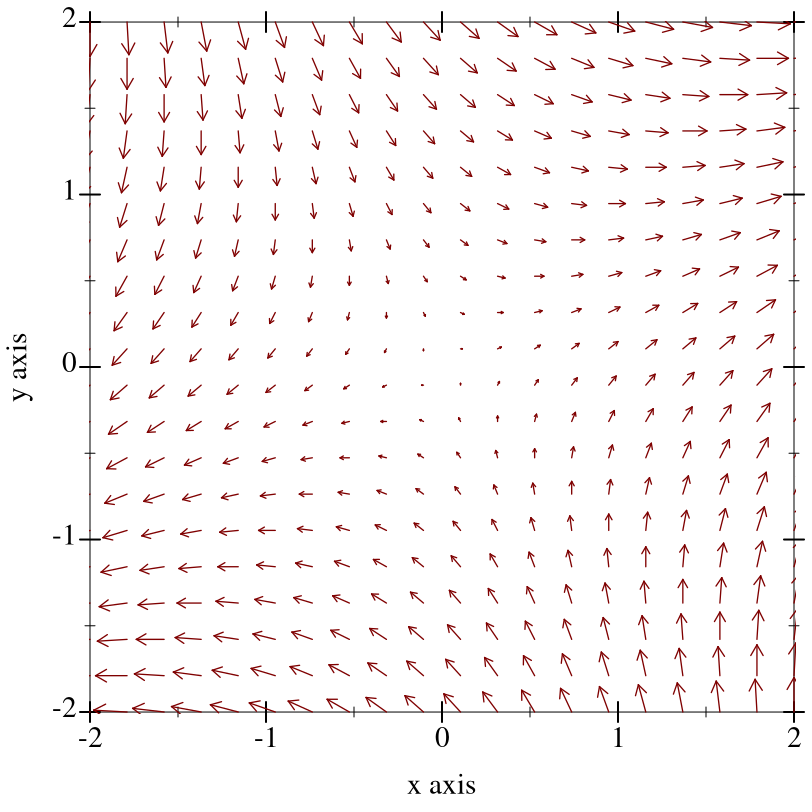Changed in version 7.9 of package plot-gui-lib: Added support for pictures for #:label and controlling the arrowhead

procedure

 (error-bars bars [ #:x-min x-min #:x-max x-max #:y-min y-min #:y-max y-max #:color color #:line-width line-width #:line-style line-style #:width width #:alpha alpha #:invert? invert?]) → renderer2d?
bars : (sequence/c (sequence/c #:min-count 3 real?))
x-min : (or/c rational? #f) = #f
x-max : (or/c rational? #f) = #f
y-min : (or/c rational? #f) = #f
y-max : (or/c rational? #f) = #f
color : plot-color/c = (error-bar-color)
line-width : (>=/c 0) = (error-bar-line-width)
line-style : plot-pen-style/c = (error-bar-line-style)
width : (>=/c 0) = (error-bar-width)
alpha : (real-in 0 1) = (error-bar-alpha)
invert? : boolean? = #f
Returns a renderer that draws error bars. The first and second element in each vector in bars comprise the coordinate; the third is the height.
 > (plot (list (function sqr 1 7) (error-bars (list (vector 2 4 12) (vector 4 16 20) (vector 6 36 10)))))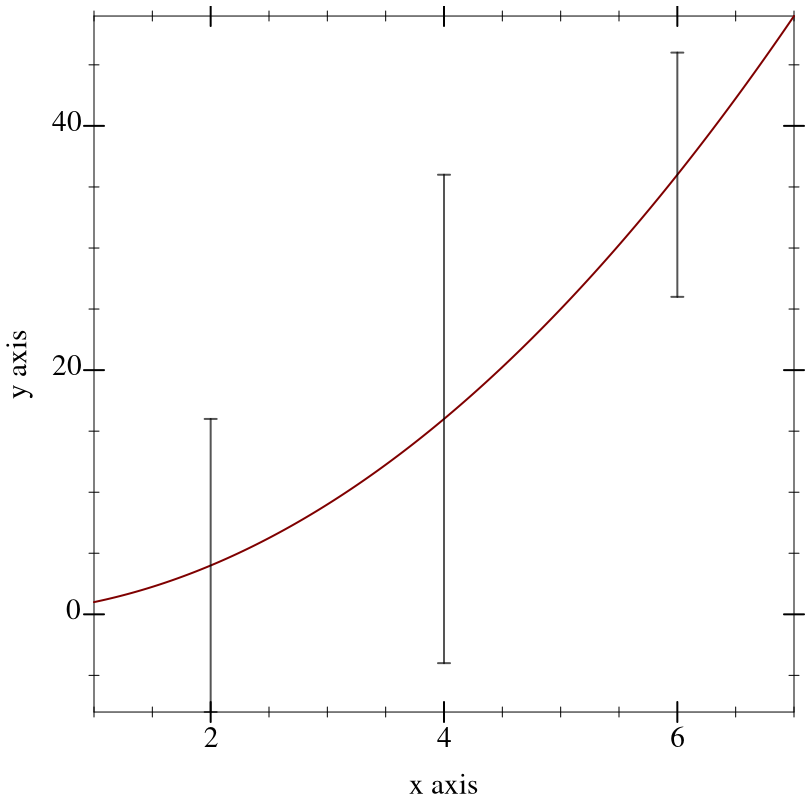If invert? is #t, the x and y coordinates are inverted, and the bars are drawn horizontally rather than vertically. This is intended for use with the corresponding option of discrete-histogram and stacked-histogram.

Changed in version 1.1 of package plot-gui-lib: Added the #:invert? option.

procedure

 (candlesticks candles [ #:x-min x-min #:x-max x-max #:y-min y-min #:y-max y-max #:up-color up-color #:down-color down-color #:line-width line-width #:line-style line-style #:width width #:alpha alpha]) → renderer2d?
candles : (sequence/c (sequence/c #:min-count 5 real?))
x-min : (or/c rational? #f) = #f
x-max : (or/c rational? #f) = #f
y-min : (or/c rational? #f) = #f
y-max : (or/c rational? #f) = #f
up-color : plot-color/c = (candlestick-up-color)
down-color : plot-color/c = (candlestick-down-color)
line-width : (>=/c 0) = (candlestick-line-width)
line-style : plot-pen-style/c = (candlestick-line-style)
width : (>=/c 0) = (candlestick-width)
alpha : (real-in 0 1) = (candlestick-alpha)
Returns a renderer that draws candlesticks. This is most common when plotting historical prices for financial instruments. The first element in each vector of candles comprises the x-axis coordinate; the second, third, fourth, and fifth elements in each vector comprise the open, high, low, and close, respectively, of the y-axis coordinates.
 > (plot (list (candlesticks (list (vector 2 4 12 4 8) (vector 4 16 20 8 12) (vector 6 24 36 10 24)))))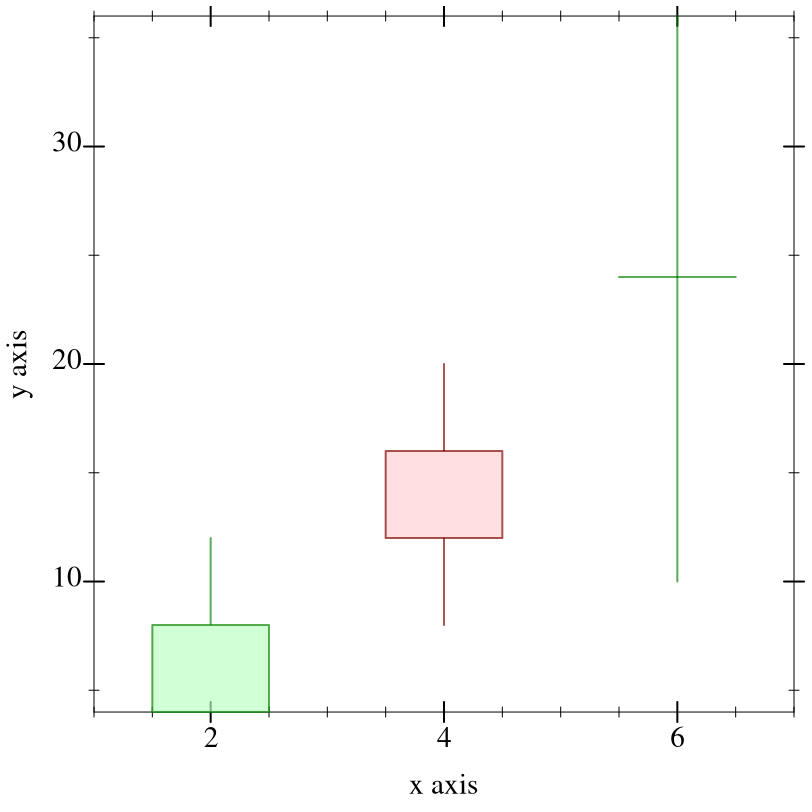procedure

 (color-field f [ x-min x-max y-min y-max #:samples samples #:alpha alpha]) → renderer2d?
f :
 (or/c (-> real? real? plot-color/c) (-> (vector/c real? real?) plot-color/c))
x-min : (or/c rational? #f) = #f
x-max : (or/c rational? #f) = #f
y-min : (or/c rational? #f) = #f
y-max : (or/c rational? #f) = #f
samples : exact-positive-integer? = (color-field-samples)
alpha : (real-in 0 1) = (color-field-alpha)
Returns a renderer that draws rectangles filled with a color based on the center point.

 > (plot (color-field (λ (x y) (if (< (+ (sqr x) (sqr y)) 1) (random 10) 'black)) -2 2 -2 2))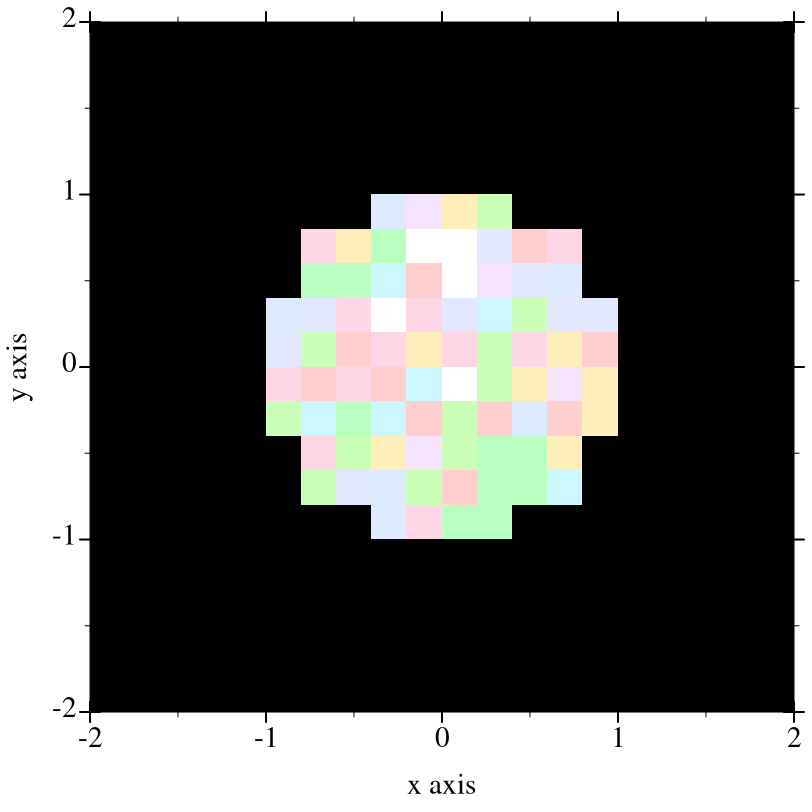Added in version 7.9 of package plot-gui-lib.

#### 3.32D Line Renderers

procedure

 (function f [ x-min x-max #:y-min y-min #:y-max y-max #:samples samples #:color color #:width width #:style style #:alpha alpha #:label label]) → renderer2d?
f : (real? . -> . real?)
x-min : (or/c rational? #f) = #f
x-max : (or/c rational? #f) = #f
y-min : (or/c rational? #f) = #f
y-max : (or/c rational? #f) = #f
samples : (and/c exact-integer? (>=/c 2)) = (line-samples)
color : plot-color/c = (line-color)
width : (>=/c 0) = (line-width)
style : plot-pen-style/c = (line-style)
alpha : (real-in 0 1) = (line-alpha)
label : (or/c string? pict? #f) = #f
Returns a renderer that plots a function of x. For example, a parabola:
 > (plot (function sqr -2 2))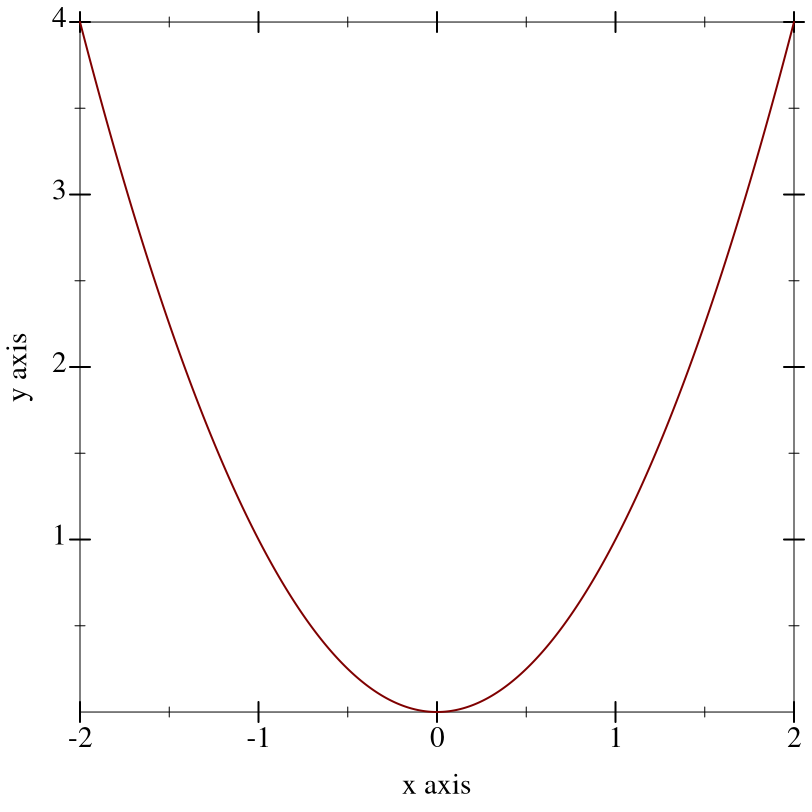Changed in version 7.9 of package plot-gui-lib: Added support for pictures for #:label

procedure

 (inverse f [ y-min y-max #:x-min x-min #:x-max x-max #:samples samples #:color color #:width width #:style style #:alpha alpha #:label label]) → renderer2d?
f : (real? . -> . real?)
y-min : (or/c rational? #f) = #f
y-max : (or/c rational? #f) = #f
x-min : (or/c rational? #f) = #f
x-max : (or/c rational? #f) = #f
samples : (and/c exact-integer? (>=/c 2)) = (line-samples)
color : plot-color/c = (line-color)
width : (>=/c 0) = (line-width)
style : plot-pen-style/c = (line-style)
alpha : (real-in 0 1) = (line-alpha)
label : (or/c string? pict? #f) = #f
Like function, but regards f as a function of y. For example, a parabola, an inverse parabola, and the reflection line:
 > (plot (list (axes) (function sqr -2 2 #:label "y = x²") (function (λ (x) x) #:color 0 #:style 'dot #:label "y = x") (inverse sqr -2 2 #:color 3 #:label "x = y²")))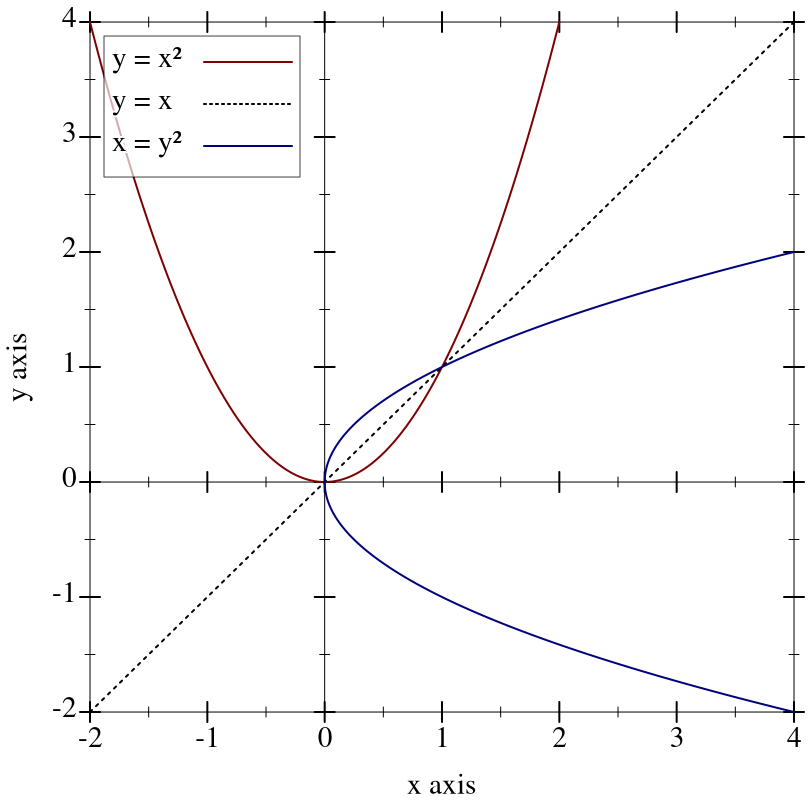Changed in version 7.9 of package plot-gui-lib: Added support for pictures for #:label

procedure

 (lines vs [ #:x-min x-min #:x-max x-max #:y-min y-min #:y-max y-max #:color color #:width width #:style style #:alpha alpha #:label label]) → renderer2d?
vs : (sequence/c (sequence/c #:min-count 2 real?))
x-min : (or/c rational? #f) = #f
x-max : (or/c rational? #f) = #f
y-min : (or/c rational? #f) = #f
y-max : (or/c rational? #f) = #f
color : plot-color/c = (line-color)
width : (>=/c 0) = (line-width)
style : plot-pen-style/c = (line-style)
alpha : (real-in 0 1) = (line-alpha)
label : (or/c string? pict? #f) = #f
Returns a renderer that draws lines connecting the points in the input sequence vs.

This is directly useful for plotting a time series, such as a random walk:

 > (plot (lines (reverse (for/fold ([lst (list (vector 0 0))]) ([i  (in-range 1 200)]) (match-define (vector x y) (first lst)) (cons (vector i (+ y (* 1/100 (- (random) 1/2)))) lst))) #:color 6 #:label "Random walk"))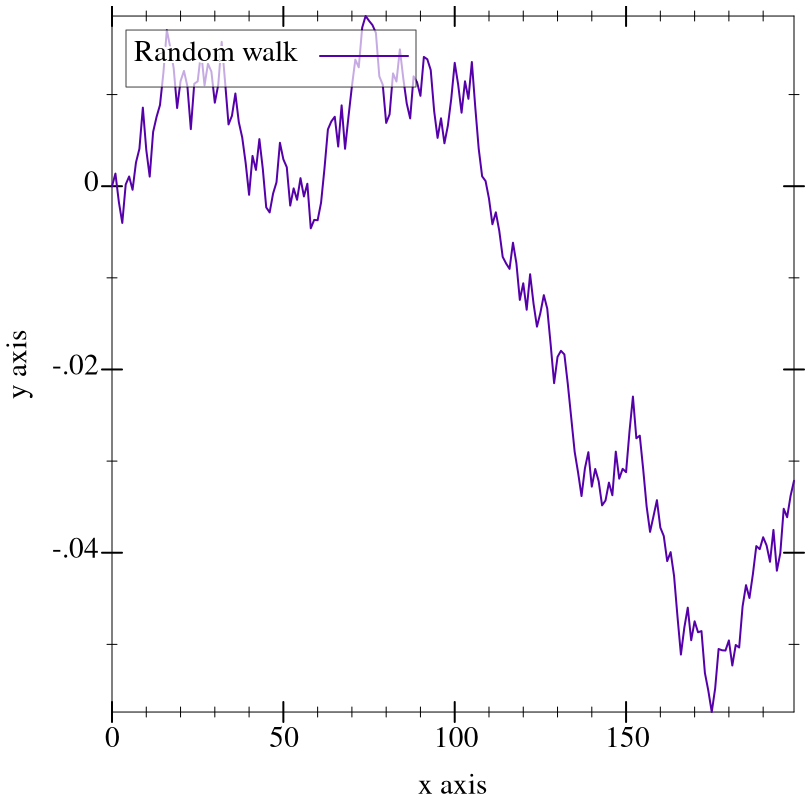The parametric and polar functions are defined using lines.

If any of the points in vs is +nan.0, no line segment will be drawn at that position. This can be used to draw several independent data sets with one lines renderer, improving rendering performence if the datasets contain a large number of points.

Changed in version 7.9 of package plot-gui-lib: Added support for pictures for #:label

procedure

 (parametric f t-min t-max [ #:x-min x-min #:x-max x-max #:y-min y-min #:y-max y-max #:samples samples #:color color #:width width #:style style #:alpha alpha #:label label]) → renderer2d?
f : (real? . -> . (sequence/c real?))
t-min : rational?
t-max : rational?
x-min : (or/c rational? #f) = #f
x-max : (or/c rational? #f) = #f
y-min : (or/c rational? #f) = #f
y-max : (or/c rational? #f) = #f
samples : (and/c exact-integer? (>=/c 2)) = (line-samples)
color : plot-color/c = (line-color)
width : (>=/c 0) = (line-width)
style : plot-pen-style/c = (line-style)
alpha : (real-in 0 1) = (line-alpha)
label : (or/c string? pict? #f) = #f
Returns a renderer that plots vector-valued functions of time. For example, the circle as a function of time can be plotted using
 > (plot (parametric (λ (t) (vector (cos t) (sin t))) 0 (* 2 pi)))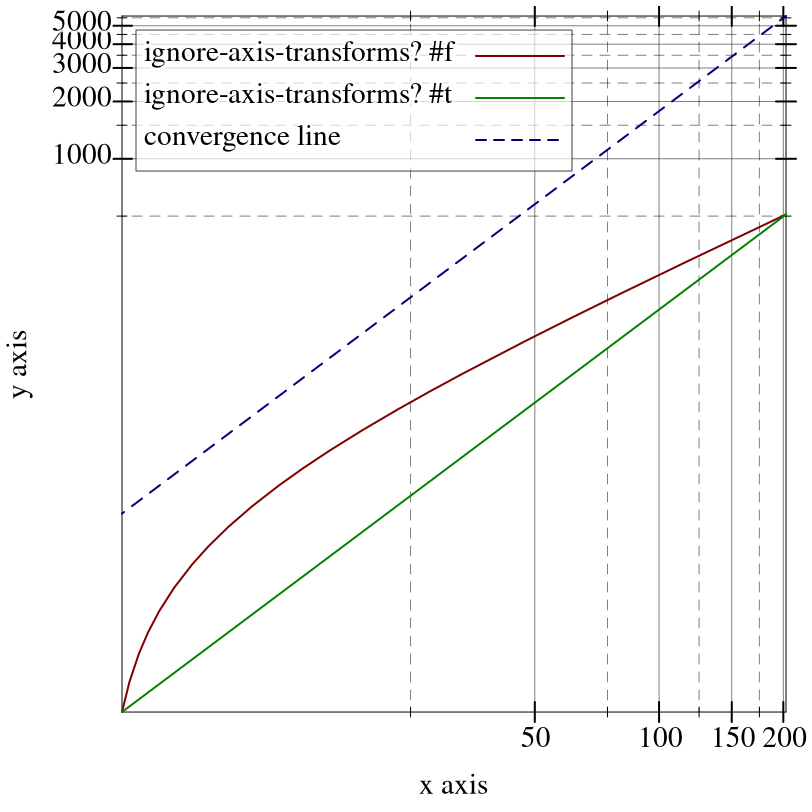Changed in version 7.9 of package plot-gui-lib: Added support for pictures for #:label

procedure

 (polar f [ θ-min θ-max #:x-min x-min #:x-max x-max #:y-min y-min #:y-max y-max #:samples samples #:color color #:width width #:style style #:alpha alpha #:label label]) → renderer2d?
f : (real? . -> . real?)
θ-min : real? = 0
θ-max : real? = (* 2 pi)
x-min : (or/c rational? #f) = #f
x-max : (or/c rational? #f) = #f
y-min : (or/c rational? #f) = #f
y-max : (or/c rational? #f) = #f
samples : (and/c exact-integer? (>=/c 2)) = (line-samples)
color : plot-color/c = (line-color)
width : (>=/c 0) = (line-width)
style : plot-pen-style/c = (line-style)
alpha : (real-in 0 1) = (line-alpha)
label : (or/c string? pict? #f) = #f
Returns a renderer that plots functions from angle to radius. Note that the angle parameters θ-min and θ-max default to 0 and (* 2 pi).

For example, drawing a full circle:
 > (plot (polar (λ (θ) 1)))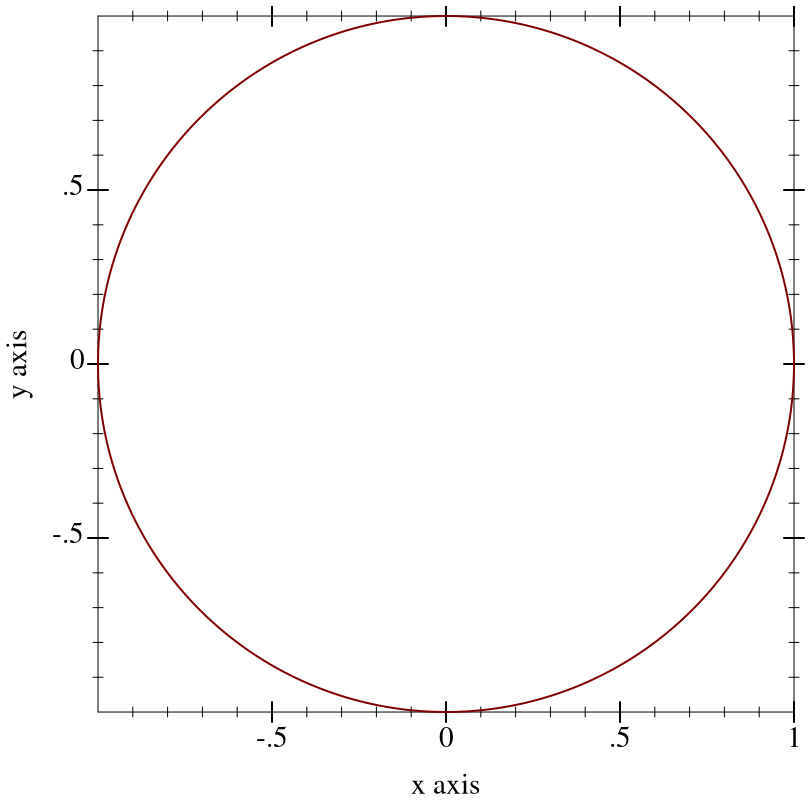Changed in version 7.9 of package plot-gui-lib: Added support for pictures for #:label

procedure

 (density xs [ bw-adjust ws #:x-min x-min #:x-max x-max #:y-min y-min #:y-max y-max #:samples samples #:color color #:width width #:style style #:alpha alpha #:label label]) → renderer2d?
xs : (sequence/c real?)
bw-adjust : (>/c 0) = 1
ws : (or/c (sequence/c (>=/c 0)) #f) = #f
x-min : (or/c rational? #f) = #f
x-max : (or/c rational? #f) = #f
y-min : (or/c rational? #f) = #f
y-max : (or/c rational? #f) = #f
samples : (and/c exact-integer? (>=/c 2)) = (line-samples)
color : plot-color/c = (line-color)
width : (>=/c 0) = (line-width)
style : plot-pen-style/c = (line-style)
alpha : (real-in 0 1) = (line-alpha)
label : (or/c string? pict? #f) = #f
Returns a renderer that plots an estimated density for the given points, which are optionally weighted by ws. The bandwidth for the kernel is calculated as (* bw-adjust 1.06 sd (expt n -0.2)), where sd is the standard deviation of the data and n is the number of points. Currently, the only supported kernel is the Gaussian.

For example, to plot an estimated density of the triangle distribution:
 > (plot (list (function (λ (x) (cond [(or (x . < . -1) (x . > . 1))  0] [(x . < . 0)   (+ 1 x)] [(x . >= . 0)  (- 1 x)])) -1.5 1.5 #:label "Density") (density (build-list 2000 (λ (n) (- (+ (random) (random)) 1))) #:color 0 #:width 2 #:style 'dot #:label "Est. density")))Changed in version 7.9 of package plot-gui-lib: Added support for pictures for #:label

procedure

 (arrows vs [ #:x-min x-min #:x-max x-max #:y-min y-min #:y-max y-max #:color color #:width width #:style style #:alpha alpha #:arrow-head-size-or-scale size #:arrow-head-angle angle #:label label]) → renderer2d?
vs :
 (or/c (listof (sequence/c #:min-count 2 real?)) (vectorof (vector/c (sequence/c #:min-count 2 real?) (sequence/c #:min-count 2 real?))))
x-min : (or/c rational? #f) = #f
x-max : (or/c rational? #f) = #f
y-min : (or/c rational? #f) = #f
y-max : (or/c rational? #f) = #f
color : plot-color/c = (arrows-color)
width : (>=/c 0) = (arrows-line-width)
style : plot-pen-style/c = (arrows-line-style)
alpha : (real-in 0 1) = (arrows-alpha)
 size : (or/c (list/c '= (>=/c 0)) (>=/c 0)) = (arrow-head-size-or-scale)
angle : (>=/c 0) = (arrow-head-angle)
label : (or/c string? pict? #f) = #f
Returns a renderer which draws arrows. Arrows can be specified either as sequences of 2D points, in this case they will be drawn as connected arrows between each two adjacent points, or they can be specified as an origin point and a rectangular magnitude vector, in which case each arrow is drawn individually. See example below.

In vs list and vector are interchangeable. Arrow-heads are only drawn when the endpoint is inside the drawing area.
 > (plot (list (arrows `((0 0) (2 1) (3 3) (0 0)) #:arrow-head-size-or-scale '(= 20) #:arrow-head-angle 0.2 #:color 6 #:label "a+b+c=0") (arrows `(((2 0) (0 1)) ((3 0) (-1 1))) #:arrow-head-size-or-scale 0.2 #:color 2 #:label "d")))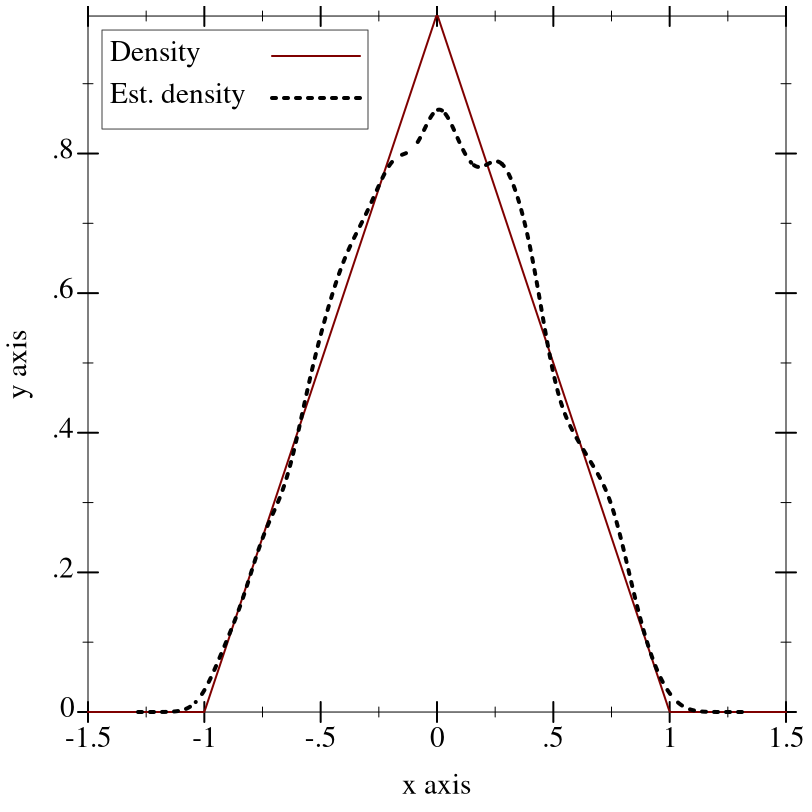Added in version 7.9 of package plot-gui-lib.

procedure

 (hrule y [ x-min x-max #:color color #:width width #:style style #:alpha alpha #:label label]) → renderer2d?
y : real?
x-min : (or/c rational? #f) = #f
x-max : (or/c rational? #f) = #f
color : plot-color/c = (line-color)
width : (>=/c 0) = (line-width)
style : plot-pen-style/c = (line-style)
alpha : (real-in 0 1) = (line-alpha)
label : (or/c string? pict? #f) = #f
Draws a horizontal line at y. By default, the line spans the entire plot area width.

Changed in version 7.9 of package plot-gui-lib: Added support for pictures for #:label

procedure

 (vrule x [ y-min y-max #:color color #:width width #:style style #:alpha alpha #:label label]) → renderer2d?
x : real?
y-min : (or/c rational? #f) = #f
y-max : (or/c rational? #f) = #f
color : plot-color/c = (line-color)
width : (>=/c 0) = (line-width)
style : plot-pen-style/c = (line-style)
alpha : (real-in 0 1) = (line-alpha)
label : (or/c string? pict? #f) = #f
Draws a vertical line at x. By default, the line spans the entire plot area height.

Changed in version 7.9 of package plot-gui-lib: Added support for pictures for #:label

#### 3.42D Interval Renderers

These renderers each correspond with a line renderer, and graph the area between two lines.

procedure

 (function-interval f1 f2 [ x-min x-max #:y-min y-min #:y-max y-max #:samples samples #:color color #:style style #:line1-color line1-color #:line1-width line1-width #:line1-style line1-style #:line2-color line2-color #:line2-width line2-width #:line2-style line2-style #:alpha alpha #:label label]) → renderer2d?
f1 : (real? . -> . real?)
f2 : (real? . -> . real?)
x-min : (or/c rational? #f) = #f
x-max : (or/c rational? #f) = #f
y-min : (or/c rational? #f) = #f
y-max : (or/c rational? #f) = #f
samples : (and/c exact-integer? (>=/c 2)) = (line-samples)
color : plot-color/c = (interval-color)
style : plot-brush-style/c = (interval-style)
line1-color : plot-color/c = (interval-line1-color)
line1-width : (>=/c 0) = (interval-line1-width)
line1-style : plot-pen-style/c = (interval-line1-style)
line2-color : plot-color/c = (interval-line2-color)
line2-width : (>=/c 0) = (interval-line2-width)
line2-style : plot-pen-style/c = (interval-line2-style)
alpha : (real-in 0 1) = (interval-alpha)
label : (or/c string? pict? #f) = #f
Corresponds with function.

 > (plot (function-interval (λ (x) 0) (λ (x) (exp (* -1/2 (sqr x)))) -4 4 #:line1-style 'transparent))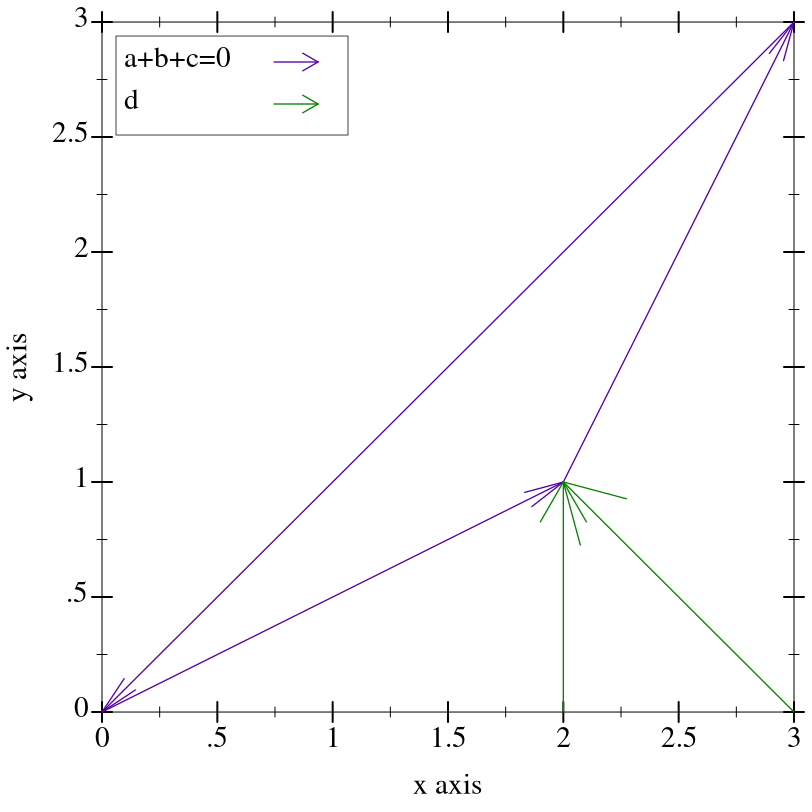Changed in version 7.9 of package plot-gui-lib: Added support for pictures for #:label

procedure

 (inverse-interval f1 f2 [ y-min y-max #:x-min x-min #:x-max x-max #:samples samples #:color color #:style style #:line1-color line1-color #:line1-width line1-width #:line1-style line1-style #:line2-color line2-color #:line2-width line2-width #:line2-style line2-style #:alpha alpha #:label label]) → renderer2d?
f1 : (real? . -> . real?)
f2 : (real? . -> . real?)
y-min : (or/c rational? #f) = #f
y-max : (or/c rational? #f) = #f
x-min : (or/c rational? #f) = #f
x-max : (or/c rational? #f) = #f
samples : (and/c exact-integer? (>=/c 2)) = (line-samples)
color : plot-color/c = (interval-color)
style : plot-brush-style/c = (interval-style)
line1-color : plot-color/c = (interval-line1-color)
line1-width : (>=/c 0) = (interval-line1-width)
line1-style : plot-pen-style/c = (interval-line1-style)
line2-color : plot-color/c = (interval-line2-color)
line2-width : (>=/c 0) = (interval-line2-width)
line2-style : plot-pen-style/c = (interval-line2-style)
alpha : (real-in 0 1) = (interval-alpha)
label : (or/c string? pict? #f) = #f
Corresponds with inverse.

 > (plot (inverse-interval sin (λ (x) 0) (- pi) pi #:line2-style 'long-dash))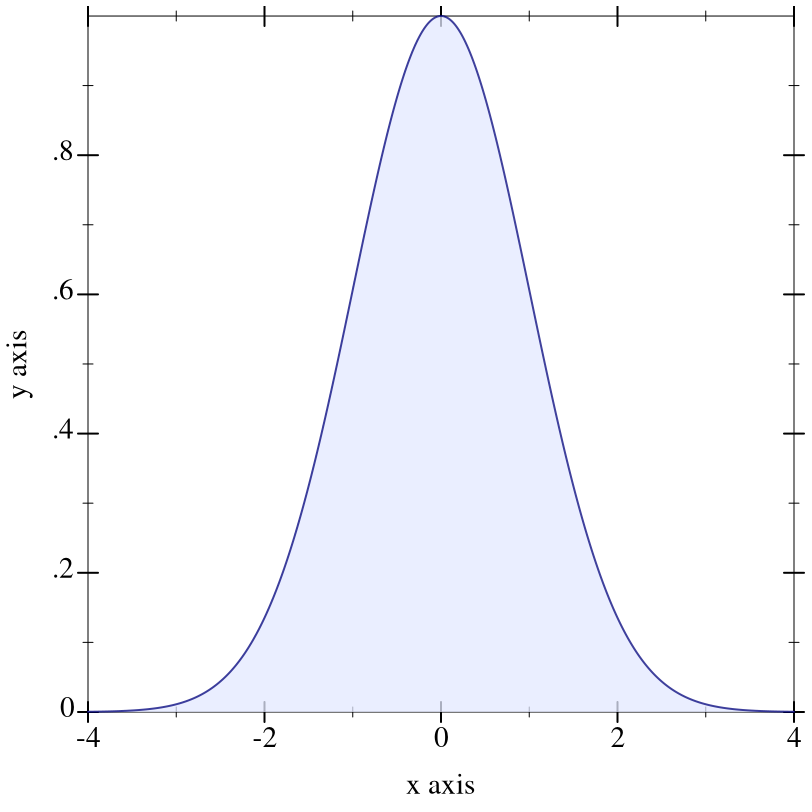Changed in version 7.9 of package plot-gui-lib: Added support for pictures for #:label

procedure

 (lines-interval v1s v2s [ #:x-min x-min #:x-max x-max #:y-min y-min #:y-max y-max #:color color #:style style #:line1-color line1-color #:line1-width line1-width #:line1-style line1-style #:line2-color line2-color #:line2-width line2-width #:line2-style line2-style #:alpha alpha #:label label]) → renderer2d?
v1s : (sequence/c (sequence/c #:min-count 2 real?))
v2s : (sequence/c (sequence/c #:min-count 2 real?))
x-min : (or/c rational? #f) = #f
x-max : (or/c rational? #f) = #f
y-min : (or/c rational? #f) = #f
y-max : (or/c rational? #f) = #f
color : plot-color/c = (interval-color)
style : plot-brush-style/c = (interval-style)
line1-color : plot-color/c = (interval-line1-color)
line1-width : (>=/c 0) = (interval-line1-width)
line1-style : plot-pen-style/c = (interval-line1-style)
line2-color : plot-color/c = (interval-line2-color)
line2-width : (>=/c 0) = (interval-line2-width)
line2-style : plot-pen-style/c = (interval-line2-style)
alpha : (real-in 0 1) = (interval-alpha)
label : (or/c string? pict? #f) = #f
Corresponds with lines.

 > (plot (list (tick-grid) (lines-interval (list #(0 0) #(1 1/2)) (list #(0 1) #(1 3/2)) #:color 4 #:line1-color 4 #:line2-color 4 #:label "Parallelogram")))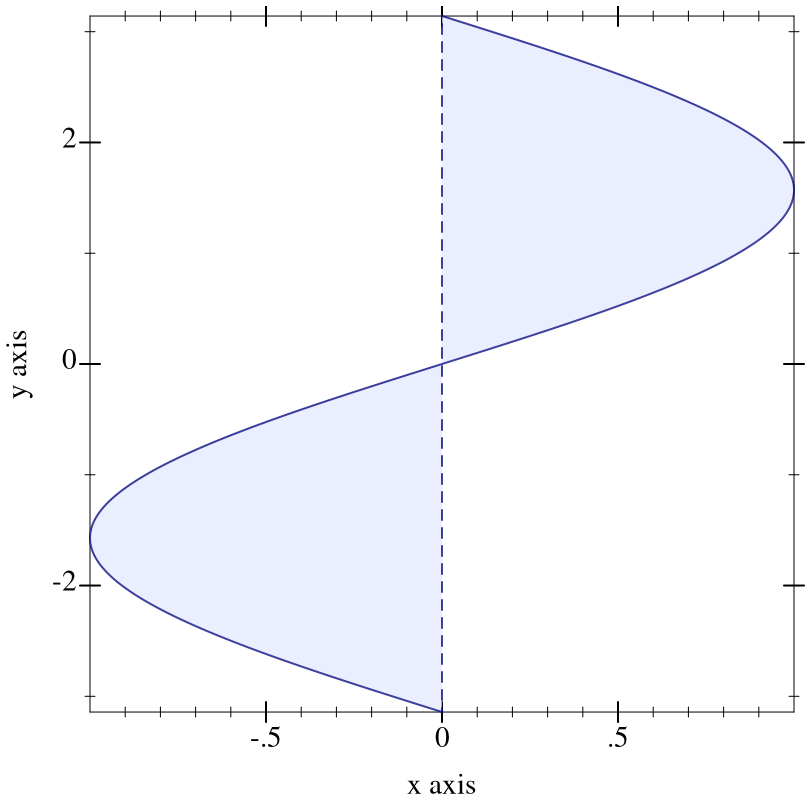Changed in version 7.9 of package plot-gui-lib: Added support for pictures for #:label

procedure

 (parametric-interval f1 f2 t-min t-max [ #:x-min x-min #:x-max x-max #:y-min y-min #:y-max y-max #:samples samples #:color color #:style style #:line1-color line1-color #:line1-width line1-width #:line1-style line1-style #:line2-color line2-color #:line2-width line2-width #:line2-style line2-style #:alpha alpha #:label label]) → renderer2d?
f1 : (real? . -> . (sequence/c real?))
f2 : (real? . -> . (sequence/c real?))
t-min : rational?
t-max : rational?
x-min : (or/c rational? #f) = #f
x-max : (or/c rational? #f) = #f
y-min : (or/c rational? #f) = #f
y-max : (or/c rational? #f) = #f
samples : (and/c exact-integer? (>=/c 2)) = (line-samples)
color : plot-color/c = (interval-color)
style : plot-brush-style/c = (interval-style)
line1-color : plot-color/c = (interval-line1-color)
line1-width : (>=/c 0) = (interval-line1-width)
line1-style : plot-pen-style/c = (interval-line1-style)
line2-color : plot-color/c = (interval-line2-color)
line2-width : (>=/c 0) = (interval-line2-width)
line2-style : plot-pen-style/c = (interval-line2-style)
alpha : (real-in 0 1) = (interval-alpha)
label : (or/c string? pict? #f) = #f
Corresponds with parametric.

 > (define (f1 t) (vector (* 2 (cos (* 4/5 t))) (* 2 (sin (* 4/5 t)))))
 > (define (f2 t) (vector (* 1/2 (cos t)) (* 1/2 (sin t))))
> (plot (parametric-interval f1 f2 (- pi) pi))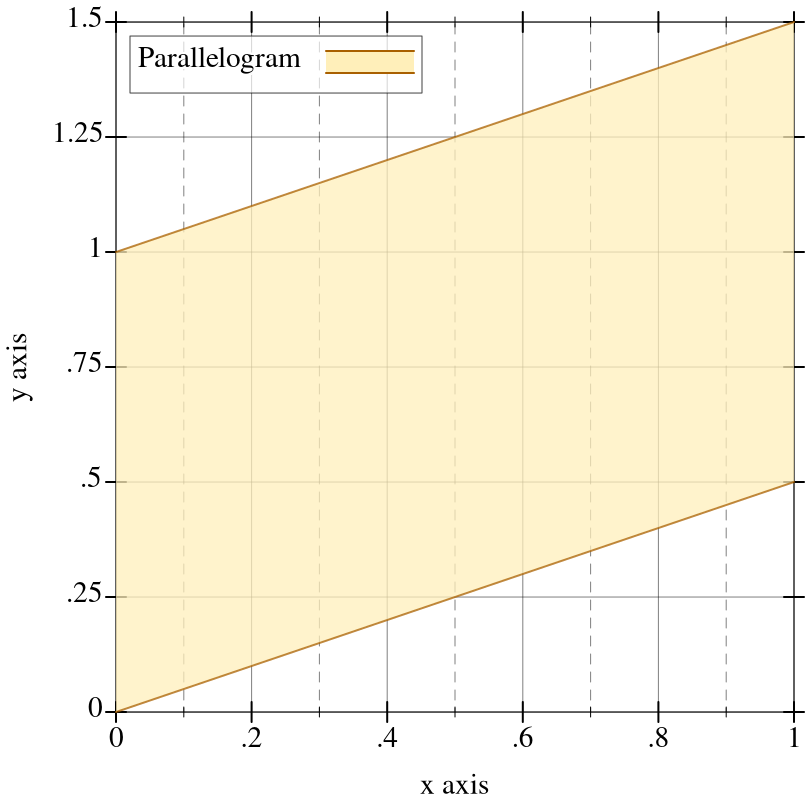Changed in version 7.9 of package plot-gui-lib: Added support for pictures for #:label

procedure

 (polar-interval f1 f2 [ θ-min θ-max #:x-min x-min #:x-max x-max #:y-min y-min #:y-max y-max #:samples samples #:color color #:style style #:line1-color line1-color #:line1-width line1-width #:line1-style line1-style #:line2-color line2-color #:line2-width line2-width #:line2-style line2-style #:alpha alpha #:label label]) → renderer2d?
f1 : (real? . -> . real?)
f2 : (real? . -> . real?)
θ-min : rational? = 0
θ-max : rational? = (* 2 pi)
x-min : (or/c rational? #f) = #f
x-max : (or/c rational? #f) = #f
y-min : (or/c rational? #f) = #f
y-max : (or/c rational? #f) = #f
samples : (and/c exact-integer? (>=/c 2)) = (line-samples)
color : plot-color/c = (interval-color)
style : plot-brush-style/c = (interval-style)
line1-color : plot-color/c = (interval-line1-color)
line1-width : (>=/c 0) = (interval-line1-width)
line1-style : plot-pen-style/c = (interval-line1-style)
line2-color : plot-color/c = (interval-line2-color)
line2-width : (>=/c 0) = (interval-line2-width)
line2-style : plot-pen-style/c = (interval-line2-style)
alpha : (real-in 0 1) = (interval-alpha)
label : (or/c string? pict? #f) = #f
Corresponds with polar.

> (define (f1 θ) (+ 1/2 (* 1/6 (cos (* 5 θ)))))
> (define (f2 θ) (+ 1 (* 1/4 (cos (* 10 θ)))))
 > (plot (list (polar-axes #:number 10) (polar-interval f1 f2 #:label "[f1,f2]")))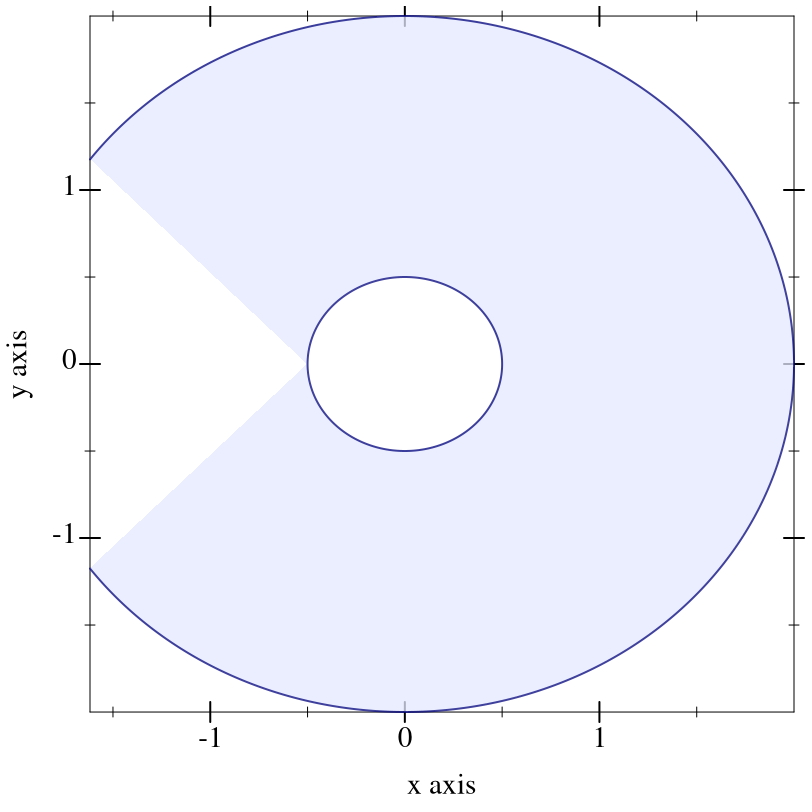Changed in version 7.9 of package plot-gui-lib: Added support for pictures for #:label

#### 3.52D Contour (Isoline) Renderers

procedure

 (isoline f z [ x-min x-max y-min y-max #:samples samples #:color color #:width width #:style style #:alpha alpha #:label label]) → renderer2d?
f : (real? real? . -> . real?)
z : real?
x-min : (or/c rational? #f) = #f
x-max : (or/c rational? #f) = #f
y-min : (or/c rational? #f) = #f
y-max : (or/c rational? #f) = #f
samples : (and/c exact-integer? (>=/c 2)) = (contour-samples)
color : plot-color/c = (line-color)
width : (>=/c 0) = (line-width)
style : plot-pen-style/c = (line-style)
alpha : (real-in 0 1) = (line-alpha)
label : (or/c string? pict? #f) = #f
Returns a renderer that plots a contour line, or a line of constant value (height). A circle of radius r, for example, is the line of constant value r for the distance function:
 > (plot (isoline (λ (x y) (sqrt (+ (sqr x) (sqr y)))) 1.5 -2 2 -2 2 #:label "z"))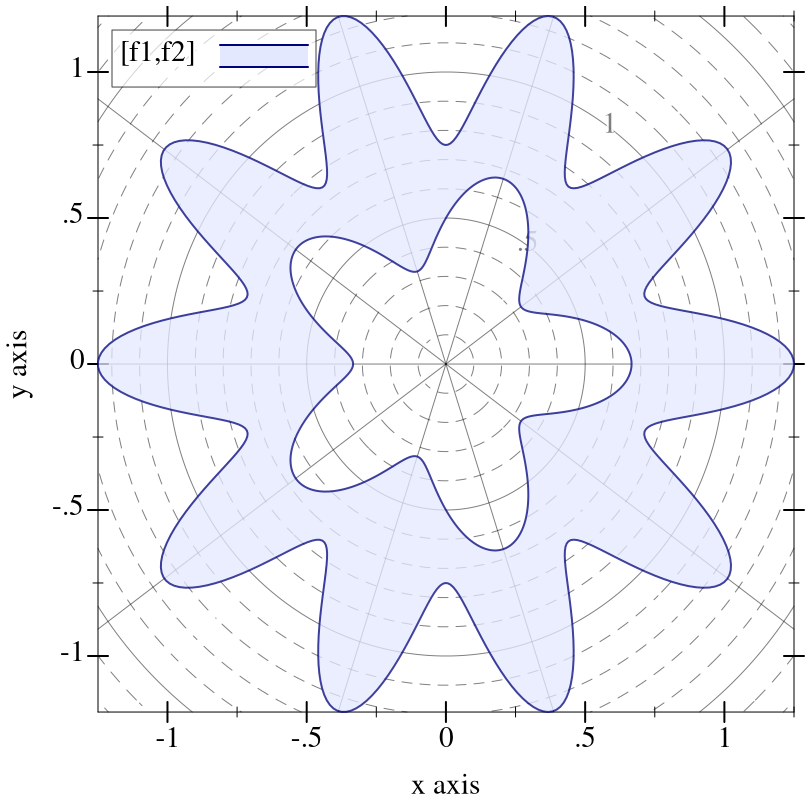Changed in version 7.9 of package plot-gui-lib: Added support for pictures for #:label

In this case, r = 1.5.

This function would have been named contour, except the name was already used by a deprecated function. It may be renamed in the future, with isoline as an alias.

procedure

 (contours f [ x-min x-max y-min y-max #:samples samples #:levels levels #:colors colors #:widths widths #:styles styles #:alphas alphas #:label label]) → renderer2d?
f : (real? real? . -> . real?)
x-min : (or/c rational? #f) = #f
x-max : (or/c rational? #f) = #f
y-min : (or/c rational? #f) = #f
y-max : (or/c rational? #f) = #f
samples : (and/c exact-integer? (>=/c 2)) = (contour-samples)
 levels : (or/c 'auto exact-positive-integer? (listof real?)) = (contour-levels)
colors : (plot-colors/c (listof real?)) = (contour-colors)
widths : (pen-widths/c (listof real?)) = (contour-widths)
styles : (plot-pen-styles/c (listof real?)) = (contour-styles)
alphas : (alphas/c (listof real?)) = (contour-alphas)
label : (or/c string? pict? #f) = #f
Returns a renderer that plots contour lines, or lines of constant value (height).

When levels is 'auto, the number of contour lines and their values are chosen the same way as axis tick positions; i.e. they are chosen to be simple. When levels is a number, contours chooses that number of values, evenly spaced, within the output range of f. When levels is a list, contours plots contours at the values in levels.

 > (plot (contours (λ (x y) (- (sqr x) (sqr y))) -2 2 -2 2 #:label "z"))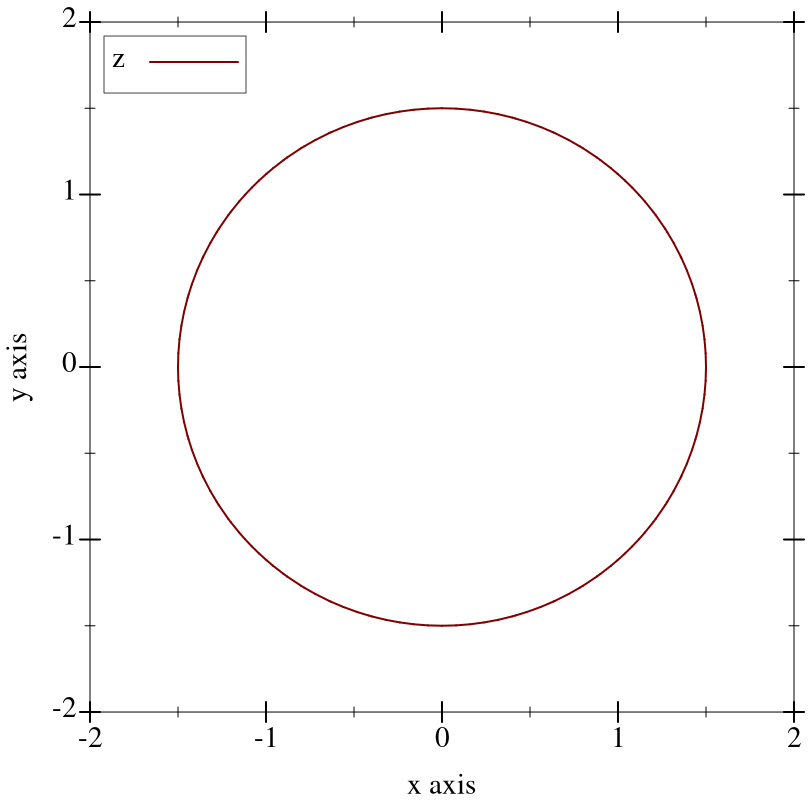The appearance keyword arguments assign a color, width, style and opacity to each contour line. Each can be given as a list or as a function from a list of output values of f to a list of appearance values. In both cases, when there are more contour lines than list elements, the colors, widths, styles and alphas in the list repeat.

For example,
 > (plot (contours (λ (x y) (- (sqr x) (sqr y))) -2 2 -2 2 #:levels 4 #:colors '("blue" "red") #:widths '(4 1) #:styles '(solid dot)))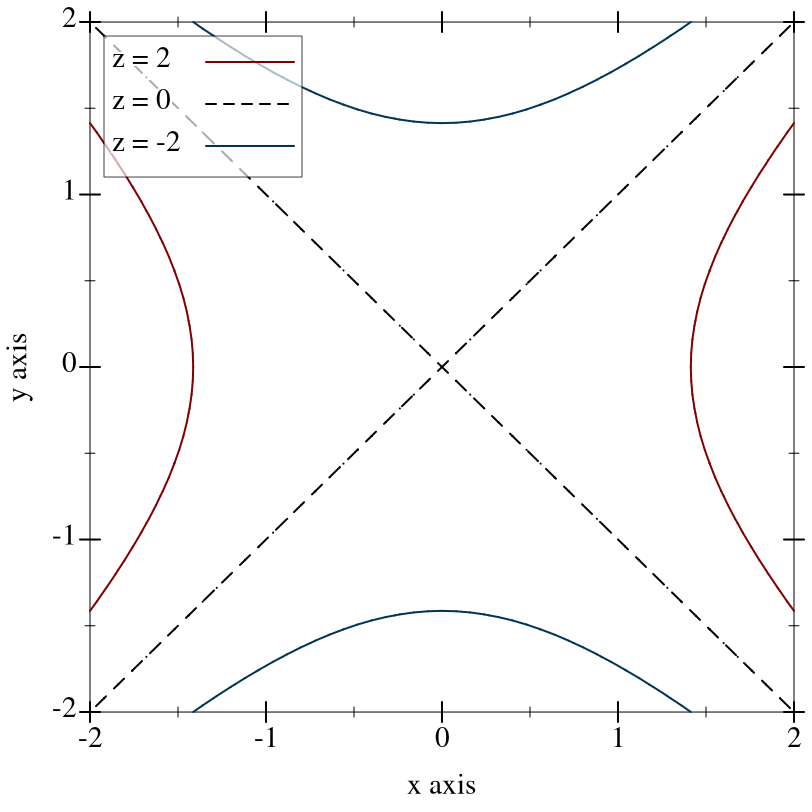Changed in version 7.9 of package plot-gui-lib: Added support for pictures for #:label

procedure

 (contour-intervals f [ x-min x-max y-min y-max #:samples samples #:levels levels #:colors colors #:styles styles #:contour-colors contour-colors #:contour-widths contour-widths #:contour-styles contour-styles #:alphas alphas #:label label])
renderer2d?
f : (real? real? . -> . real?)
x-min : (or/c rational? #f) = #f
x-max : (or/c rational? #f) = #f
y-min : (or/c rational? #f) = #f
y-max : (or/c rational? #f) = #f
samples : (and/c exact-integer? (>=/c 2)) = (contour-samples)
 levels : (or/c 'auto exact-positive-integer? (listof real?)) = (contour-levels)
 colors : (plot-colors/c (listof ivl?)) = (contour-interval-colors)
 styles : (plot-brush-styles/c (listof ivl?)) = (contour-interval-styles)
 contour-colors : (plot-colors/c (listof real?)) = (contour-colors)
 contour-widths : (pen-widths/c (listof real?)) = (contour-widths)
 contour-styles : (plot-pen-styles/c (listof real?)) = (contour-styles)
alphas : (alphas/c (listof ivl?)) = (contour-interval-alphas)
label : (or/c string? pict? #f) = #f
Returns a renderer that fills the area between contour lines, and additionally draws contour lines.

 > (plot (list (contour-intervals (λ (x y) (- (sqr x) (sqr y))) -2 2 -2 2 #:label "z") (vector-field (λ (x y) (vector (* 2 x) (* -2 y))) #:color "black" #:label "Gradient")))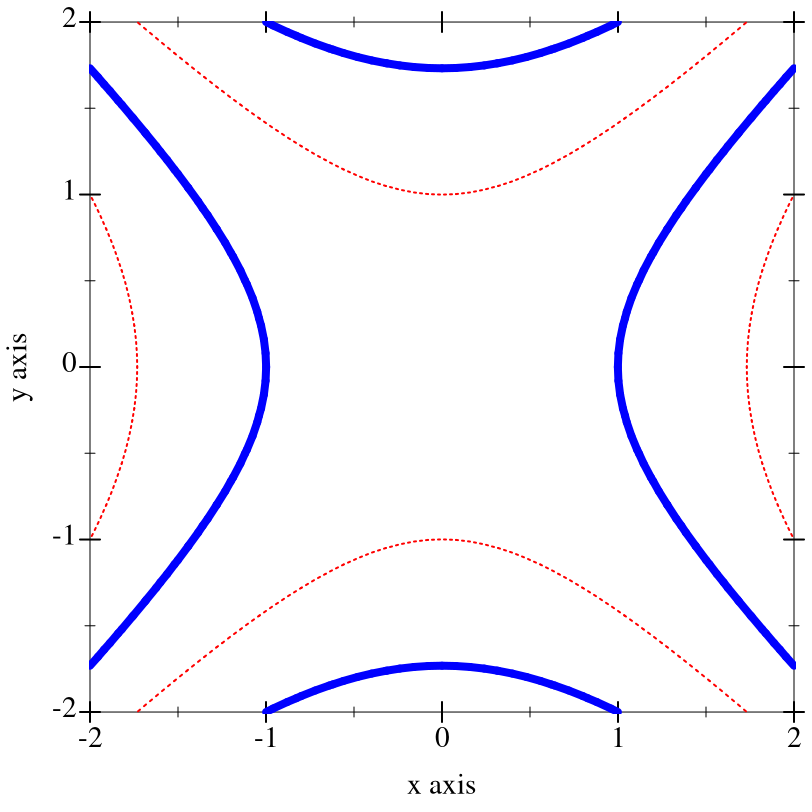Changed in version 7.9 of package plot-gui-lib: Added support for pictures for #:label

#### 3.62D Rectangle Renderers

procedure

 (rectangles rects [ #:x-min x-min #:x-max x-max #:y-min y-min #:y-max y-max #:color color #:style style #:line-color line-color #:line-width line-width #:line-style line-style #:alpha alpha #:label label]) → renderer2d?
rects : (sequence/c (sequence/c #:min-count 2 ivl?))
x-min : (or/c rational? #f) = #f
x-max : (or/c rational? #f) = #f
y-min : (or/c rational? #f) = #f
y-max : (or/c rational? #f) = #f
color : plot-color/c = (rectangle-color)
style : plot-brush-style/c = (rectangle-style)
line-color : plot-color/c = (rectangle-line-color)
line-width : (>=/c 0) = (rectangle-line-width)
line-style : plot-pen-style/c = (rectangle-line-style)
alpha : (real-in 0 1) = (rectangle-alpha)
label : (or/c string? pict? #f) = #f
Returns a renderer that draws rectangles.

The rectangles are given as a sequence of sequences of intervals—each inner sequence defines the bounds of a rectangle. Any of the bounds can be -inf.0 or +inf.0, in which case the rectangle extents to the edge of the plot area in the respective direction.

For example,
 > (plot (rectangles (list (vector (ivl -1 0) (ivl -1 1)) (vector (ivl 0 2) (ivl 1 2)))))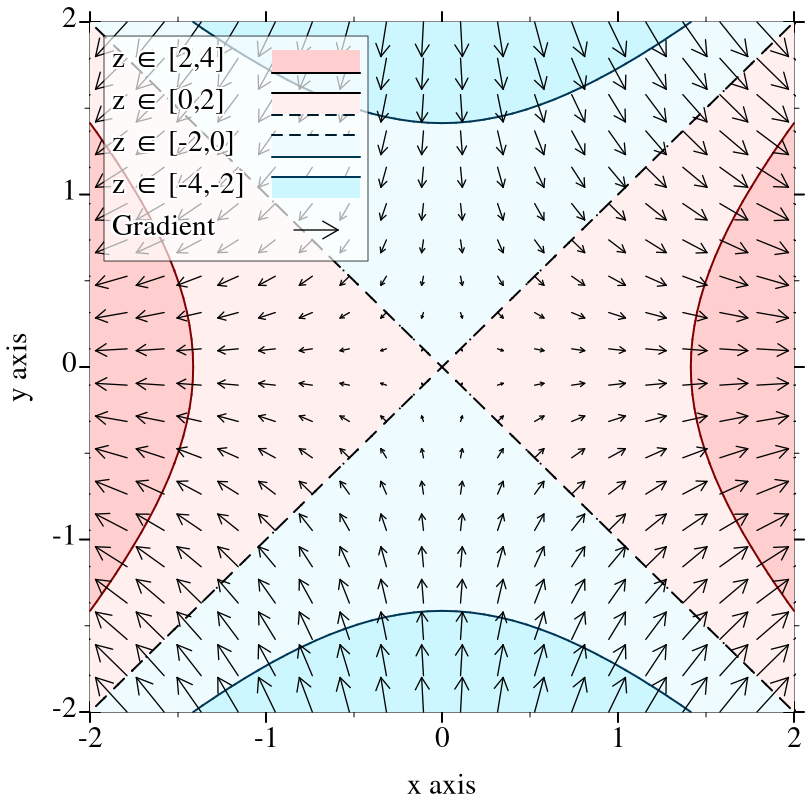Changed in version 7.9 of package plot-gui-lib: Added support for pictures for #:label

procedure

 (area-histogram f bin-bounds [ #:x-min x-min #:x-max x-max #:y-min y-min #:y-max y-max #:samples samples #:color color #:style style #:line-color line-color #:line-width line-width #:line-style line-style #:alpha alpha #:label label]) → renderer2d?
f : (real? . -> . real?)
bin-bounds : (sequence/c real?)
x-min : (or/c rational? #f) = #f
x-max : (or/c rational? #f) = #f
y-min : (or/c rational? #f) = 0
y-max : (or/c rational? #f) = #f
samples : (and/c exact-integer? (>=/c 2)) = (line-samples)
color : plot-color/c = (rectangle-color)
style : plot-brush-style/c = (rectangle-style)
line-color : plot-color/c = (rectangle-line-color)
line-width : (>=/c 0) = (rectangle-line-width)
line-style : plot-pen-style/c = (rectangle-line-style)
alpha : (real-in 0 1) = (rectangle-alpha)
label : (or/c string? pict? #f) = #f
Returns a renderer that draws a histogram approximating the area under a curve. The #:samples argument determines the accuracy of the calculated areas.
> (require (only-in plot/utils linear-seq))
> (define (f x) (exp (* -1/2 (sqr x))))
 > (plot (list (area-histogram f (linear-seq -4 4 10)) (function f -4 4)))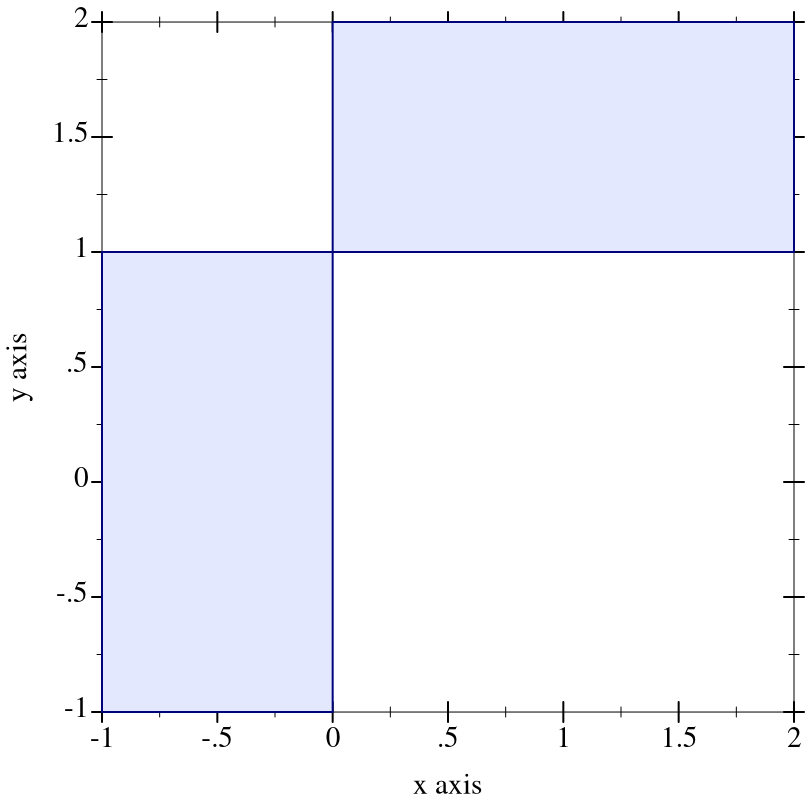procedure

 (discrete-histogram cat-vals [ #:x-min x-min #:x-max x-max #:y-min y-min #:y-max y-max #:gap gap #:skip skip #:invert? invert? #:color color #:style style #:line-color line-color #:line-width line-width #:line-style line-style #:alpha alpha #:label label #:add-ticks? add-ticks? #:far-ticks? far-ticks?]) → renderer2d?
cat-vals :
 (sequence/c (or/c (vector/c any/c (or/c real? ivl? #f)) (list/c any/c (or/c real? ivl? #f))))
x-min : (or/c rational? #f) = 0
x-max : (or/c rational? #f) = #f
y-min : (or/c rational? #f) = 0
y-max : (or/c rational? #f) = #f
gap : (real-in 0 1) = (discrete-histogram-gap)
skip : (>=/c 0) = (discrete-histogram-skip)
invert? : boolean? = (discrete-histogram-invert?)
color : plot-color/c = (rectangle-color)
style : plot-brush-style/c = (rectangle-style)
line-color : plot-color/c = (rectangle-line-color)
line-width : (>=/c 0) = (rectangle-line-width)
line-style : plot-pen-style/c = (rectangle-line-style)
alpha : (real-in 0 1) = (rectangle-alpha)
label : (or/c string? pict? #f) = #f
far-ticks? : boolean? = #f
Returns a renderer that draws a discrete histogram.

Example:
 > (plot (discrete-histogram (list #(A 1) #(B 2) #(B 3) (vector 'C (ivl 0.5 1.5)))))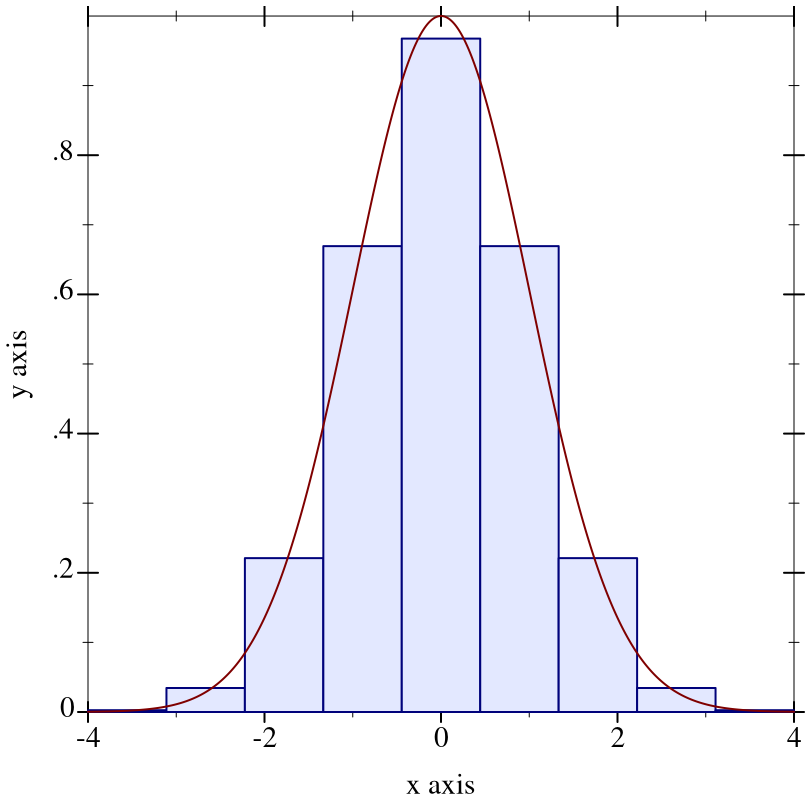Use #:invert? #t to draw horizontal bars. See stacked-histogram for an example.

Each bar takes up exactly one plot unit, and is drawn with (* 1/2 gap) empty space on each side. Bar number i is drawn at (+ x-min (* i skip)). Thus, the first bar (i = 0) is drawn in the interval between x-min (default 0) and (+ x-min 1).

To plot two histograms side-by-side, pass the appropriate x-min value to the second renderer. For example,
 > (plot (list (discrete-histogram (list #(a 1) #(b 2) #(c 3) #(d 2) #(e 4) #(f 2.5) #(g 1)) #:label "Numbers per letter") (discrete-histogram (list #(1 1) #(4 2) #(3 1.5)) #:x-min 8 #:label "Numbers per number" #:color 2 #:line-color 2)))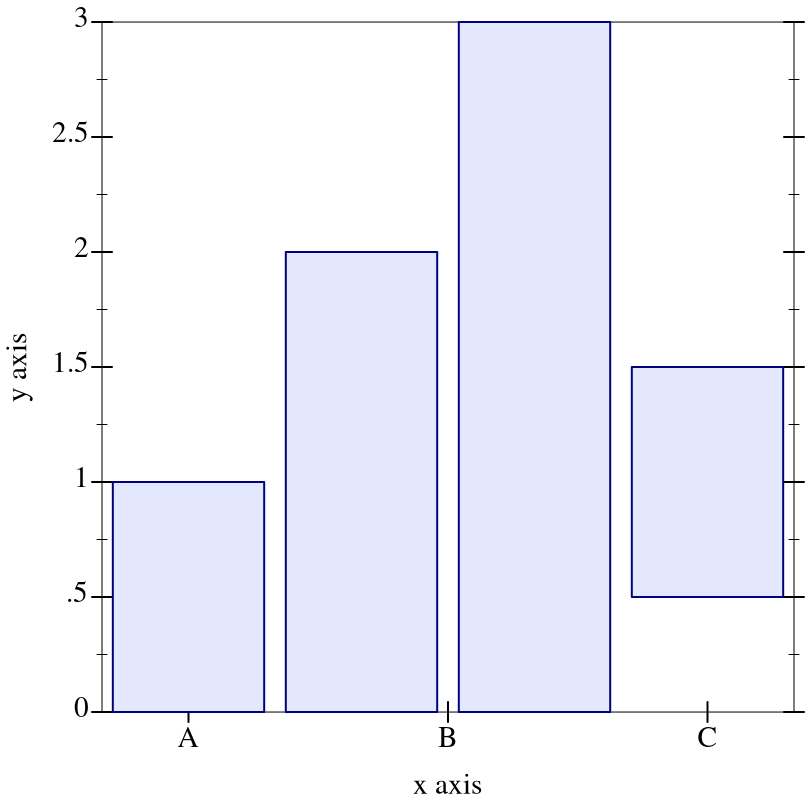Here, the first histogram has 7 bars, so the second is drawn starting at x-min = 8.

To interleave histograms, such as when plotting benchmark results, use a skip value larger than or equal to the number of histograms, and give each histogram a different x-min. For example,
 > (plot (list (discrete-histogram '(#(Eggs 1.5) #(Bacon 2.5) #(Pancakes 3.5)) #:skip 2.5 #:x-min 0 #:label "AMD") (discrete-histogram '(#(Eggs 1.4) #(Bacon 2.3) #(Pancakes 3.1)) #:skip 2.5 #:x-min 1 #:label "Intel" #:color 2 #:line-color 2)) #:x-label "Breakfast Food" #:y-label "Cooking Time (minutes)" #:title "Cooking Times For Breakfast Food, Per Processor")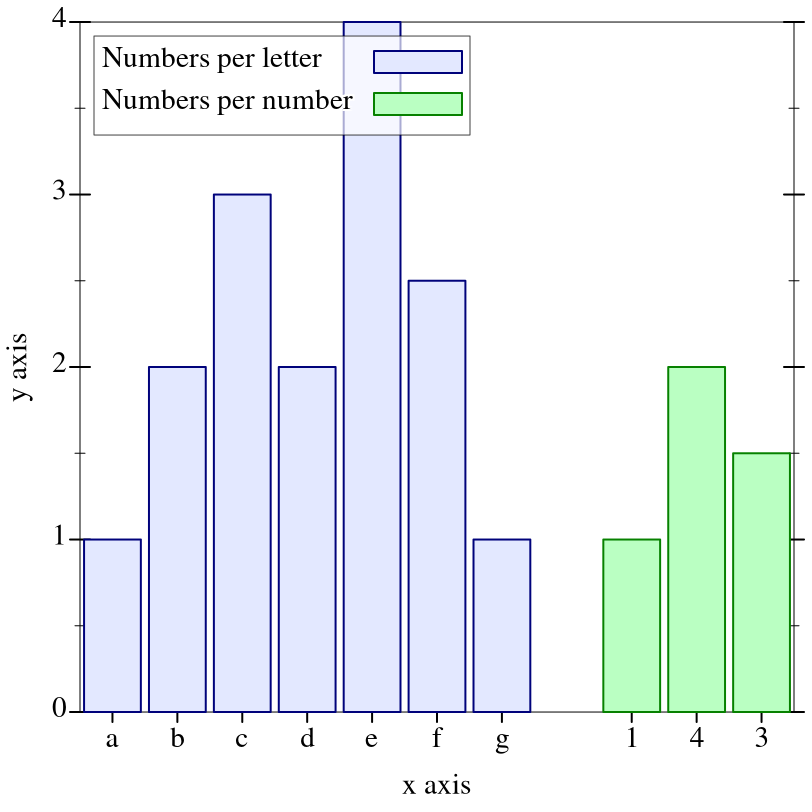When interleaving many histograms, consider setting the discrete-histogram-skip parameter to change skip’s default value.

Changed in version 7.9 of package plot-gui-lib: Added support for pictures for #:label

procedure

 (stacked-histogram cat-vals [ #:x-min x-min #:x-max x-max #:y-min y-min #:y-max y-max #:gap gap #:skip skip #:invert? invert? #:colors colors #:styles styles #:line-colors line-colors #:line-widths line-widths #:line-styles line-styles #:alphas alphas #:labels labels #:add-ticks? add-ticks? #:far-ticks? far-ticks?])
(listof renderer2d?)
cat-vals :
 (sequence/c (or/c (vector/c any/c (sequence/c real?)) (list/c any/c (sequence/c real?))))
x-min : (or/c rational? #f) = 0
x-max : (or/c rational? #f) = #f
y-min : (or/c rational? #f) = 0
y-max : (or/c rational? #f) = #f
gap : (real-in 0 1) = (discrete-histogram-gap)
skip : (>=/c 0) = (discrete-histogram-skip)
invert? : boolean? = (discrete-histogram-invert?)
colors : (plot-colors/c nat/c) = (stacked-histogram-colors)
 styles : (plot-brush-styles/c nat/c) = (stacked-histogram-styles)
 line-colors : (plot-colors/c nat/c) = (stacked-histogram-line-colors)
 line-widths : (pen-widths/c nat/c) = (stacked-histogram-line-widths)
 line-styles : (plot-pen-styles/c nat/c) = (stacked-histogram-line-styles)
alphas : (alphas/c nat/c) = (stacked-histogram-alphas)
labels : (labels/c nat/c) = '(#f)
far-ticks? : boolean? = #f
Returns a list of renderers that draw parts of a stacked histogram. The heights of each bar section are given as a list.

Example:
 > (plot (stacked-histogram (list #(a (1 1 1)) #(b (1.5 3)) #(c ()) #(d (1/2))) #:invert? #t #:labels '("Red" #f "Blue")) #:legend-anchor 'top-right)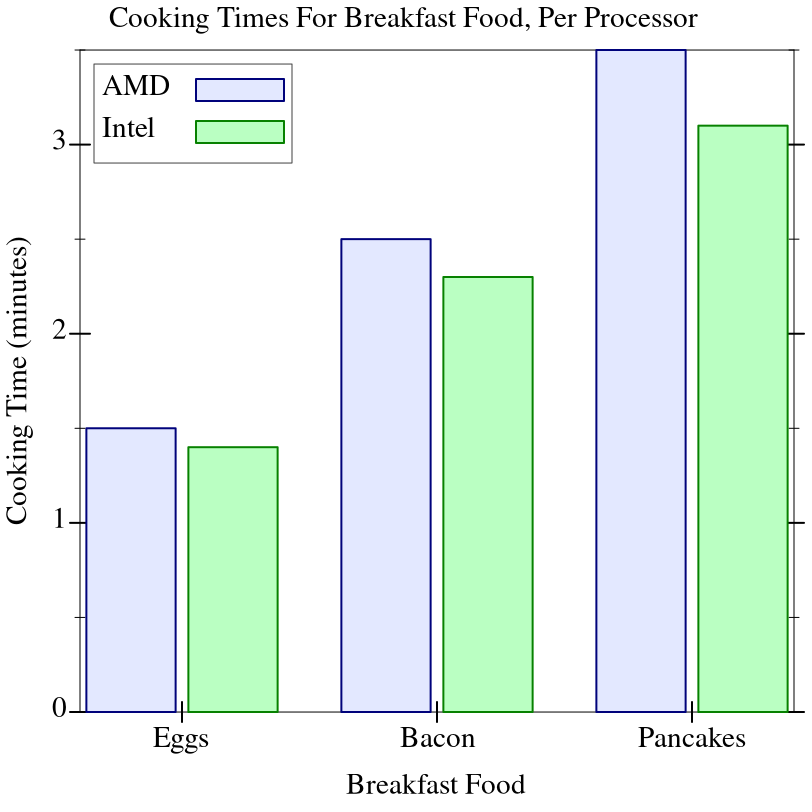#### 3.72D Plot Decoration Renderers

procedure

 (x-axis [ y #:ticks? ticks? #:labels? labels? #:far? far? #:alpha alpha]) → renderer2d?
y : real? = 0
ticks? : boolean? = (x-axis-ticks?)
labels? : boolean? = (x-axis-labels?)
far? : boolean? = (x-axis-far?)
alpha : (real-in 0 1) = (x-axis-alpha)
Returns a renderer that draws an x axis.

procedure

 (y-axis [ x #:ticks? ticks? #:labels? labels? #:far? far? #:alpha alpha]) → renderer2d?
x : real? = 0
ticks? : boolean? = (y-axis-ticks?)
labels? : boolean? = (y-axis-labels?)
far? : boolean? = (y-axis-far?)
alpha : (real-in 0 1) = (y-axis-alpha)
Returns a renderer that draws a y axis.

procedure

 (axes [ x y #:x-ticks? x-ticks? #:y-ticks? y-ticks? #:x-labels? x-labels? #:y-labels? y-labels? #:x-alpha x-alpha #:y-alpha y-alpha]) → (listof renderer2d?)
x : real? = 0
y : real? = 0
x-ticks? : boolean? = (x-axis-ticks?)
y-ticks? : boolean? = (y-axis-ticks?)
x-labels? : boolean? = (x-axis-labels?)
y-labels? : boolean? = (y-axis-labels?)
x-alpha : (real-in 0 1) = (x-axis-alpha)
y-alpha : (real-in 0 1) = (y-axis-alpha)
Returns a list containing an x-axis renderer and a y-axis renderer. See inverse for an example.

procedure

 (polar-axes [ #:number num #:ticks? ticks? #:labels? labels? #:alpha alpha]) → renderer2d?
num : exact-nonnegative-integer? = (polar-axes-number)
ticks? : boolean? = (polar-axes-ticks?)
labels? : boolean? = (polar-axes-labels?)
alpha : (real-in 0 1) = (polar-axes-alpha)
Returns a renderer that draws polar axes. See polar-interval for an example.

 procedure
Returns a renderer that draws vertical lines from the lower x-axis ticks to the upper.

 procedure
Returns a renderer that draws horizontal lines from the left y-axis ticks to the right.

 procedure
Returns a list containing an x-tick-lines renderer and a y-tick-lines renderer. See lines-interval for an example.

procedure

 (point-label v [ label #:color color #:size size #:face face #:family family #:anchor anchor #:angle angle #:point-color point-color #:point-fill-color point-fill-color #:point-size point-size #:point-line-width point-line-width #:point-sym point-sym #:alpha alpha]) → renderer2d?
v : (sequence/c real?)
label : (or/c string? #f) = #f
color : plot-color/c = (plot-foreground)
size : (>=/c 0) = (plot-font-size)
face : (or/c string? #f) = (plot-font-face)
family : font-family/c = (plot-font-family)
anchor : anchor/c = (label-anchor)
angle : real? = (label-angle)
point-color : plot-color/c = (point-color)
point-fill-color : (or/c plot-color/c 'auto) = 'auto
point-size : (>=/c 0) = (label-point-size)
point-line-width : (>=/c 0) = (point-line-width)
point-sym : point-sym/c = 'fullcircle
alpha : (real-in 0 1) = (label-alpha)
Returns a renderer that draws a labeled point. If label is #f, the point is labeled with its position.

 > (plot (list (function sqr 0 2) (point-label (vector 1 1))))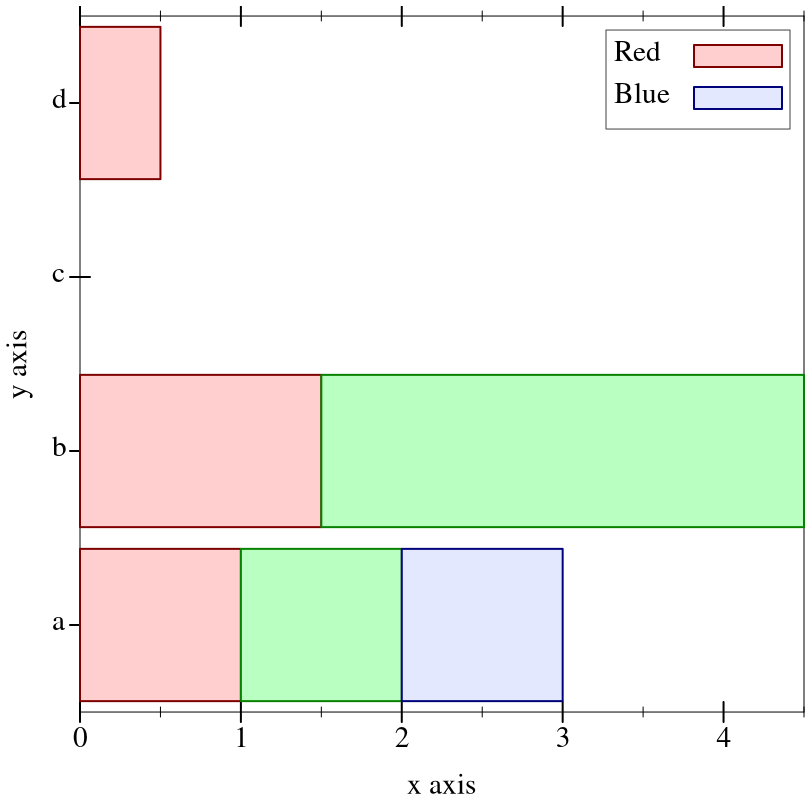The remaining labeled-point functions are defined in terms of this one.

procedure

 (point-pict v pict [ #:anchor anchor #:point-color point-color #:point-fill-color point-fill-color #:point-size point-size #:point-line-width point-line-width #:point-sym point-sym #:alpha alpha]) → renderer2d?
v : (sequence/c real?)
pict : pict?
anchor : anchor/c = (label-anchor)
point-color : plot-color/c = (point-color)
point-fill-color : (or/c plot-color/c 'auto) = 'auto
point-size : (>=/c 0) = (label-point-size)
point-line-width : (>=/c 0) = (point-line-width)
point-sym : point-sym/c = 'fullcircle
alpha : (real-in 0 1) = (label-alpha)
Returns a renderer that draws a point with a pict as the label.

> (require pict)
 > (plot (list (function sqr 0 2) (point-pict (vector 1 1) (standard-fish 40 15))))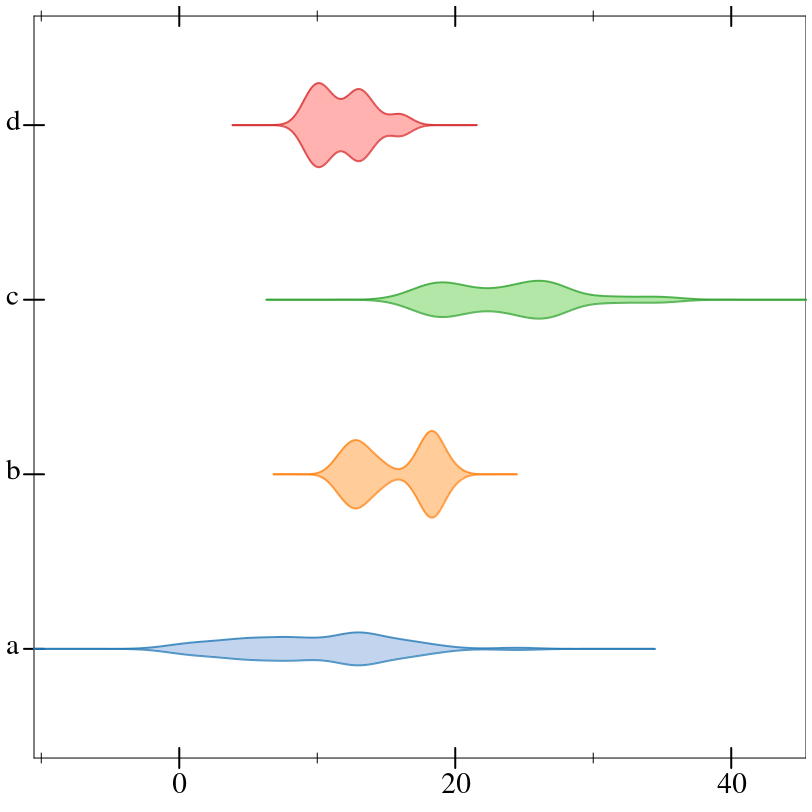The remaining labeled-pict functions are defined in terms of this one.

procedure

 (function-label f x [ label #:color color #:size size #:face face #:family family #:anchor anchor #:angle angle #:point-color point-color #:point-fill-color point-fill-color #:point-size point-size #:point-line-width point-line-width #:point-sym point-sym #:alpha alpha])
renderer2d?
f : (real? . -> . real?)
x : real?
label : (or/c string? #f) = #f
color : plot-color/c = (plot-foreground)
size : (>=/c 0) = (plot-font-size)
face : (or/c string? #f) = (plot-font-face)
family : font-family/c = (plot-font-family)
anchor : anchor/c = (label-anchor)
angle : real? = (label-angle)
point-color : plot-color/c = (point-color)
point-fill-color : (or/c plot-color/c 'auto) = 'auto
point-size : (>=/c 0) = (label-point-size)
point-line-width : (>=/c 0) = (point-line-width)
point-sym : point-sym/c = 'fullcircle
alpha : (real-in 0 1) = (label-alpha)
Returns a renderer that draws a labeled point on a function’s graph.

 > (plot (list (function sin (- pi) pi) (function-label sin (* 1/6 pi) "(1/6 π, 1/2)" #:anchor 'right)))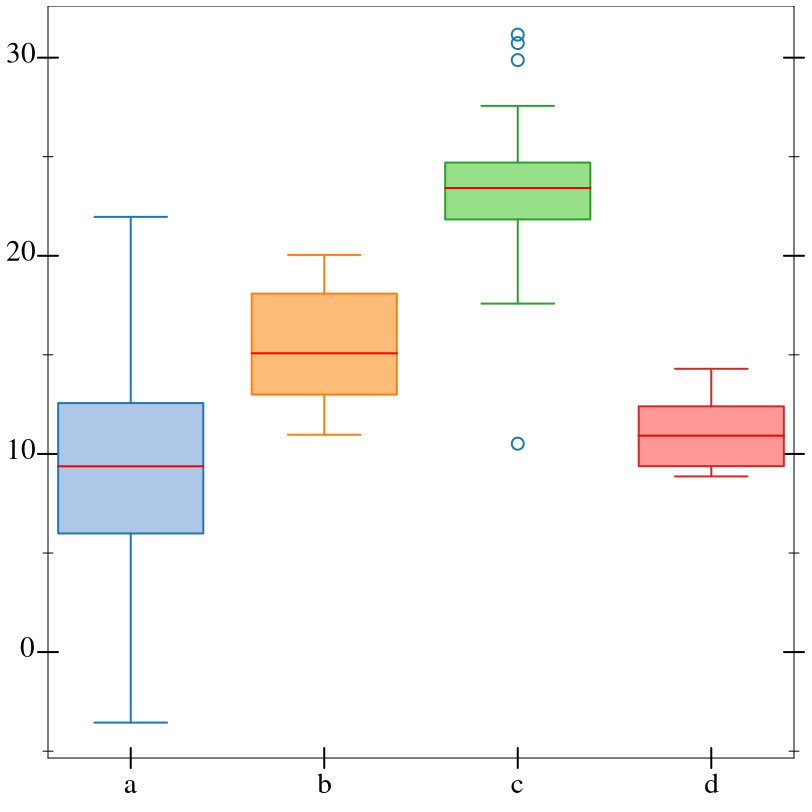procedure

 (function-pict f x pict [ #:anchor anchor #:point-color point-color #:point-fill-color point-fill-color #:point-size point-size #:point-line-width point-line-width #:point-sym point-sym #:alpha alpha])
renderer2d?
f : (real? . -> . real?)
x : real?
pict : pict?
anchor : anchor/c = (label-anchor)
point-color : plot-color/c = (point-color)
point-fill-color : (or/c plot-color/c 'auto) = 'auto
point-size : (>=/c 0) = (label-point-size)
point-line-width : (>=/c 0) = (point-line-width)
point-sym : point-sym/c = 'fullcircle
alpha : (real-in 0 1) = (label-alpha)
Returns a renderer that draws a point with a pict as the label on a function’s graph.

procedure

 (inverse-label f y [ label #:color color #:size size #:face face #:family family #:anchor anchor #:angle angle #:point-color point-color #:point-fill-color point-fill-color #:point-size point-size #:point-line-width point-line-width #:point-sym point-sym #:alpha alpha])
renderer2d?
f : (real? . -> . real?)
y : real?
label : (or/c string? #f) = #f
color : plot-color/c = (plot-foreground)
size : (>=/c 0) = (plot-font-size)
face : (or/c string? #f) = (plot-font-face)
family : font-family/c = (plot-font-family)
anchor : anchor/c = (label-anchor)
angle : real? = (label-angle)
point-color : plot-color/c = (point-color)
point-fill-color : (or/c plot-color/c 'auto) = 'auto
point-size : (>=/c 0) = (label-point-size)
point-line-width : (>=/c 0) = (point-line-width)
point-sym : point-sym/c = 'fullcircle
alpha : (real-in 0 1) = (label-alpha)
Returns a renderer that draws a labeled point on a function’s inverted graph.

procedure

 (inverse-pict f y pict [ #:anchor anchor #:point-color point-color #:point-fill-color point-fill-color #:point-size point-size #:point-line-width point-line-width #:point-sym point-sym #:alpha alpha])
renderer2d?
f : (real? . -> . real?)
y : real?
pict : pict?
anchor : anchor/c = (label-anchor)
point-color : plot-color/c = (point-color)
point-fill-color : (or/c plot-color/c 'auto) = 'auto
point-size : (>=/c 0) = (label-point-size)
point-line-width : (>=/c 0) = (point-line-width)
point-sym : point-sym/c = 'fullcircle
alpha : (real-in 0 1) = (label-alpha)
Returns a renderer that draws a point with a pict as the label on a function’s inverted graph.

procedure

 (parametric-label f t [ label #:color color #:size size #:face face #:family family #:anchor anchor #:angle angle #:point-color point-color #:point-fill-color point-fill-color #:point-size point-size #:point-line-width point-line-width #:point-sym point-sym #:alpha alpha])
renderer2d?
f : (real? . -> . (sequence/c real?))
t : real?
label : (or/c string? #f) = #f
color : plot-color/c = (plot-foreground)
size : (>=/c 0) = (plot-font-size)
face : (or/c string? #f) = (plot-font-face)
family : font-family/c = (plot-font-family)
anchor : anchor/c = (label-anchor)
angle : real? = (label-angle)
point-color : plot-color/c = (point-color)
point-fill-color : (or/c plot-color/c 'auto) = 'auto
point-size : (>=/c 0) = (label-point-size)
point-line-width : (>=/c 0) = (point-line-width)
point-sym : point-sym/c = 'fullcircle
alpha : (real-in 0 1) = (label-alpha)
Returns a renderer that draws a labeled point on a parametric function’s graph.

procedure

 (parametric-pict f t pict [ #:anchor anchor #:point-color point-color #:point-fill-color point-fill-color #:point-size point-size #:point-line-width point-line-width #:point-sym point-sym #:alpha alpha])
renderer2d?
f : (real? . -> . (sequence/c real?))
t : real?
pict : pict?
anchor : anchor/c = (label-anchor)
point-color : plot-color/c = (point-color)
point-fill-color : (or/c plot-color/c 'auto) = 'auto
point-size : (>=/c 0) = (label-point-size)
point-line-width : (>=/c 0) = (point-line-width)
point-sym : point-sym/c = 'fullcircle
alpha : (real-in 0 1) = (label-alpha)
Returns a renderer that draws a point with a pict as the label on a parametric function’s graph.

procedure

 (polar-label f θ [ label #:color color #:size size #:face face #:family family #:anchor anchor #:angle angle #:point-color point-color #:point-fill-color point-fill-color #:point-size point-size #:point-line-width point-line-width #:point-sym point-sym #:alpha alpha]) → renderer2d?
f : (real? . -> . real?)
θ : real?
label : (or/c string? #f) = #f
color : plot-color/c = (plot-foreground)
size : (>=/c 0) = (plot-font-size)
face : (or/c string? #f) = (plot-font-face)
family : font-family/c = (plot-font-family)
anchor : anchor/c = (label-anchor)
angle : real? = (label-angle)
point-color : plot-color/c = (point-color)
point-fill-color : (or/c plot-color/c 'auto) = 'auto
point-size : (>=/c 0) = (label-point-size)
point-line-width : (>=/c 0) = (point-line-width)
point-sym : point-sym/c = 'fullcircle
alpha : (real-in 0 1) = (label-alpha)
Returns a renderer that draws a labeled point on a polar function’s graph.

procedure

 (polar-pict f θ pict [ #:anchor anchor #:point-color point-color #:point-fill-color point-fill-color #:point-size point-size #:point-line-width point-line-width #:point-sym point-sym #:alpha alpha]) → renderer2d?
f : (real? . -> . real?)
θ : real?
pict : pict?
anchor : anchor/c = (label-anchor)
point-color : plot-color/c = (point-color)
point-fill-color : (or/c plot-color/c 'auto) = 'auto
point-size : (>=/c 0) = (label-point-size)
point-line-width : (>=/c 0) = (point-line-width)
point-sym : point-sym/c = 'fullcircle
alpha : (real-in 0 1) = (label-alpha)
Returns a renderer that draws a point with a pict as the label on a polar function’s graph.

#### 3.8Interactive Overlays for 2D plots

 (require plot/snip) package: plot-gui-lib

A plot snip% object returned by plot-snip can be set up to provide interactive overlays. This feature can be used, for example, to show the current value of the plot function at the mouse cursor.

If the code below is evaluated in DrRacket, the resulting plot will show a vertical line tracking the mouse and the current plot position is shown on a label. This is achieved by adding a mouse callback to the plot snip returned by plot-snip. When the mouse callback is invoked, it will add a vrule at the current X position and a point-label at the current value of the plotted function.

 (require plot) (define snip (plot-snip (function sin) #:x-min -5 #:x-max 5)) (define (mouse-callback snip event x y) (if (and x y) (send snip set-overlay-renderers (list (vrule x) (point-label (vector x (sin x))))) (send snip set-overlay-renderers #f))) (send snip set-mouse-event-callback mouse-callback) snip

Here are a few hints for adding common interactive elements to racket plots:

• The hrule and vrule renderers can be used to draw horizontal and vertical lines that track the mouse position

• The rectangles renderer can be used to highlight a region on the plot. For example, to highlight a vertical region between xmin and xmax, you can use:

 (rectangles (list (vector (ivl xmin xmax) (ivl -inf.0 +inf.0))) #:alpha 0.2)
• A point-label renderer can be used to add a point with a string label to the plot. To add only the label, use 'none as the value for the #:point-sym argument.

• A point-pict renderer can be used to add a point with an attached pict instead of a string label. This can be used to draw fancy labels (for example with rounded corners), or any other type of graphics element.

• A points renderer can be used to mark specific locations on the plot, without specifying a label for them

 class superclass: snip%
An instance of this class is returned by plot-snip.

 method(send a-2d-plot-snip set-mouse-event-callback callback) → any/c callback : (or/c plot-mouse-event-callback/c #f)
Set a callback function to be invoked with mouse events from the snip. The callback is invoked with the actual snip object, the mouse-event% and the X, Y position of the mouse in plot coordinates (i.e., the coordinate system used by the renderers in the plot). The X and Y values are #f when the mouse is outside the plot area (for example, when the mouse is over the axis area).

When a callback is installed, the default zoom functionality of the plot snips is disabled. This can be restored by calling set-mouse-event-callback with a #f argument.

 method(send a-2d-plot-snip set-overlay-renderers renderers) → any/c renderers : (or/c (treeof renderer2d?) #f)
Set a collection of renderers to be drawn on top of the existing plot. This can be any combination of 2D renderers, but it will not be able to modify the axes or the dimensions of the plot area. Only one set of overlay renderers can be installed; calling this method a second time will replace the previous overlays. Specifying #f as the renderers will cause overlays to be disabled.

value

=
 (-> (is-a?/c snip%) (is-a?/c mouse-event%) (or/c real? #f) (or/c real? #f) any/c)
A contract for callback functions passed to set-mouse-event-callback.